# class TH1: public TNamed, public TAttLine, public TAttFill, public TAttMarker

```
```

## The Histogram classes

ROOT supports the following histogram types:
• 1-D histograms:
• TH1C : histograms with one byte per channel. Maximum bin content = 127
• TH1S : histograms with one short per channel. Maximum bin content = 32767
• TH1I : histograms with one int per channel. Maximum bin content = 2147483647
• TH1F : histograms with one float per channel. Maximum precision 7 digits
• TH1D : histograms with one double per channel. Maximum precision 14 digits
• 2-D histograms:
• TH2C : histograms with one byte per channel. Maximum bin content = 127
• TH2S : histograms with one short per channel. Maximum bin content = 32767
• TH2I : histograms with one int per channel. Maximum bin content = 2147483647
• TH2F : histograms with one float per channel. Maximum precision 7 digits
• TH2D : histograms with one double per channel. Maximum precision 14 digits
• 3-D histograms:
• TH3C : histograms with one byte per channel. Maximum bin content = 127
• TH3S : histograms with one short per channel. Maximum bin content = 32767
• TH3I : histograms with one int per channel. Maximum bin content = 2147483647
• TH3F : histograms with one float per channel. Maximum precision 7 digits
• TH3D : histograms with one double per channel. Maximum precision 14 digits
• Profile histograms: See classes TProfile and TProfile2D. Profile histograms are used to display the mean value of Y and its RMS for each bin in X. Profile histograms are in many cases an elegant replacement of two-dimensional histograms : the inter-relation of two measured quantities X and Y can always be visualized by a two-dimensional histogram or scatter-plot; If Y is an unknown (but single-valued) approximate function of X, this function is displayed by a profile histogram with much better precision than by a scatter-plot.
All histogram classes are derived from the base class TH1
```                                TH1
^
|
|
|
-----------------------------------------------------------
|                |       |      |      |     |     |
|                |      TH1C   TH1S   TH1I  TH1F  TH1D
|                |                                 |
|                |                                 |
|               TH2                             TProfile
|                |
|                |
|                ----------------------------------
|                        |      |      |     |     |
|                       TH2C   TH2S   TH2I  TH2F  TH2D
|                                                  |
TH3                                                 |
|                                               TProfile2D
|
-------------------------------------
|      |      |      |      |
TH3C   TH3S   TH3I   TH3F   TH3D
The TH*C classes also inherit from the array class TArrayC.
The TH*S classes also inherit from the array class TArrayS.
The TH*I classes also inherit from the array class TArrayI.
The TH*F classes also inherit from the array class TArrayF.
The TH*D classes also inherit from the array class TArrayD.
```

#### Creating histograms

Histograms are created by invoking one of the constructors, eg

```       TH1F *h1 = new TH1F("h1","h1 title",100,0,4.4);
TH2F *h2 = new TH2F("h2","h2 title",40,0,4,30,-3,3);
```

Histograms may also be created by:

• calling the Clone function, see below
• making a projection from a 2-D or 3-D histogram, see below
• reading an histogram from a file

When an histogram is created, a reference to it is automatically added to the list of in-memory objects for the current file or directory. This default behaviour can be changed by:

```       h->SetDirectory(0);          for the current histogram h
TH1::AddDirectory(kFALSE);   sets a global switch disabling the reference
```
When the histogram is deleted, the reference to it is removed from the list of objects in memory. When a file is closed, all histograms in memory associated with this file are automatically deleted.

#### Fix or variable bin size

All histogram types support either fix or variable bin sizes. 2-D histograms may have fix size bins along X and variable size bins along Y or vice-versa. The functions to fill, manipulate, draw or access histograms are identical in both cases.

Each histogram always contains 3 objects TAxis: fXaxis, fYaxis and fZaxis To access the axis parameters, do:

```        TAxis *xaxis = h->GetXaxis(); etc.
Double_t binCenter = xaxis->GetBinCenter(bin), etc.
```
See class TAxis for a description of all the access functions. The axis range is always stored internally in double precision.

#### Convention for numbering bins

For all histogram types: nbins, xlow, xup
```        bin = 0;       underflow bin
bin = 1;       first bin with low-edge xlow INCLUDED
bin = nbins;   last bin with upper-edge xup EXCLUDED
bin = nbins+1; overflow bin
```

In case of 2-D or 3-D histograms, a "global bin" number is defined. For example, assuming a 3-D histogram with binx,biny,binz, the function

```        Int_t gbin = h->GetBin(binx,biny,binz);
```
returns a global/linearized gbin number. This global gbin is useful to access the bin content/error information independently of the dimension. Note that to access the information other than bin content and errors one should use the TAxis object directly with eg:
```         Double_t xcenter = h3->GetZaxis()->GetBinCenter(27);
```
returns the center along z of bin number 27 (not the global bin) in the 3-d histogram h3.

#### Alphanumeric Bin Labels

By default, an histogram axis is drawn with its numeric bin labels. One can specify alphanumeric labels instead with:
• call TAxis::SetBinLabel(bin,label); This can always be done before or after filling. When the histogram is drawn, bin labels will be automatically drawn. See example in \$ROOTSYS/tutorials/graphs/labels1.C, labels2.C
• call to a Fill function with one of the arguments being a string, eg
```           hist1->Fill(somename,weigth);
hist2->Fill(x,somename,weight);
hist2->Fill(somename,y,weight);
hist2->Fill(somenamex,somenamey,weight);
```
See example in \$ROOTSYS/tutorials/hist/hlabels1.C, hlabels2.C
• via TTree::Draw. see for example \$ROOTSYS/tutorials/tree/cernstaff.C
```           tree.Draw("Nation::Division");
```
where "Nation" and "Division" are two branches of a Tree.

When using the options 2 or 3 above, the labels are automatically added to the list (THashList) of labels for a given axis. By default, an axis is drawn with the order of bins corresponding to the filling sequence. It is possible to reorder the axis - alphabetically - by increasing or decreasing values The reordering can be triggered via the TAxis contextMenu by selecting the menu item "LabelsOption" or by calling directly TH1::LabelsOption(option,axis) where

• axis may be "X","Y" or "Z"
• option may be:
• "a" sort by alphabetic order
• ">" sort by decreasing values
• "<" sort by increasing values
• "h" draw labels horizonthal
• "v" draw labels vertical
• "u" draw labels up (end of label right adjusted)
• "d" draw labels down (start of label left adjusted)

When using the option 2 above, new labels are added by doubling the current number of bins in case one label does not exist yet. When the Filling is terminated, it is possible to trim the number of bins to match the number of active labels by calling

```           TH1::LabelsDeflate(axis) with axis = "X","Y" or "Z"
```
This operation is automatic when using TTree::Draw. Once bin labels have been created, they become persistent if the histogram is written to a file or when generating the C++ code via SavePrimitive.

#### Histograms with automatic bins

When an histogram is created with an axis lower limit greater or equal to its upper limit, the SetBuffer is automatically called with an argument fBufferSize equal to fgBufferSize (default value=1000). fgBufferSize may be reset via the static function TH1::SetDefaultBufferSize. The axis limits will be automatically computed when the buffer will be full or when the function BufferEmpty is called.

#### Filling histograms

An histogram is typically filled with statements like:
```       h1->Fill(x);
h1->Fill(x,w); fill with weight
h2->Fill(x,y)
h2->Fill(x,y,w)
h3->Fill(x,y,z)
h3->Fill(x,y,z,w)
```
or via one of the Fill functions accepting names described above. The Fill functions compute the bin number corresponding to the given x,y or z argument and increment this bin by the given weight. The Fill functions return the bin number for 1-D histograms or global bin number for 2-D and 3-D histograms.

If TH1::Sumw2 has been called before filling, the sum of squares of weights is also stored. One can also increment directly a bin number via TH1::AddBinContent or replace the existing content via TH1::SetBinContent. To access the bin content of a given bin, do:

```       Double_t binContent = h->GetBinContent(bin);
```

By default, the bin number is computed using the current axis ranges. If the automatic binning option has been set via

```       h->SetBit(TH1::kCanRebin);
```
then, the Fill Function will automatically extend the axis range to accomodate the new value specified in the Fill argument. The method used is to double the bin size until the new value fits in the range, merging bins two by two. This automatic binning options is extensively used by the TTree::Draw function when histogramming Tree variables with an unknown range.

This automatic binning option is supported for 1-d, 2-D and 3-D histograms. During filling, some statistics parameters are incremented to compute the mean value and Root Mean Square with the maximum precision.

In case of histograms of type TH1C, TH1S, TH2C, TH2S, TH3C, TH3S a check is made that the bin contents do not exceed the maximum positive capacity (127 or 32767). Histograms of all types may have positive or/and negative bin contents.

#### Rebinning

At any time, an histogram can be rebinned via TH1::Rebin. This function returns a new histogram with the rebinned contents. If bin errors were stored, they are recomputed during the rebinning.

#### Associated errors

By default, for each bin, the sum of weights is computed at fill time. One can also call TH1::Sumw2 to force the storage and computation of the sum of the square of weights per bin. If Sumw2 has been called, the error per bin is computed as the sqrt(sum of squares of weights), otherwise the error is set equal to the sqrt(bin content). To return the error for a given bin number, do:
```        Double_t error = h->GetBinError(bin);
```

#### Associated functions

One or more object (typically a TF1*) can be added to the list of functions (fFunctions) associated to each histogram. When TH1::Fit is invoked, the fitted function is added to this list. Given an histogram h, one can retrieve an associated function with:
```        TF1 *myfunc = h->GetFunction("myfunc");
```

#### Operations on histograms

Many types of operations are supported on histograms or between histograms
• Addition of an histogram to the current histogram
• Additions of two histograms with coefficients and storage into the current histogram
• Multiplications and Divisions are supported in the same way as additions.
• The Add, Divide and Multiply functions also exist to add,divide or multiply an histogram by a function.
If an histogram has associated error bars (TH1::Sumw2 has been called), the resulting error bars are also computed assuming independent histograms. In case of divisions, Binomial errors are also supported. One can mark a histogram to be an "average" histogram by setting its bit kIsAverage via myhist.SetBit(TH1::kIsAverage); When adding (see TH1::Add) average histograms, the histograms are averaged and not summed.

#### Fitting histograms

Histograms (1-D,2-D,3-D and Profiles) can be fitted with a user specified function via TH1::Fit. When an histogram is fitted, the resulting function with its parameters is added to the list of functions of this histogram. If the histogram is made persistent, the list of associated functions is also persistent. Given a pointer (see above) to an associated function myfunc, one can retrieve the function/fit parameters with calls such as:
```       Double_t chi2 = myfunc->GetChisquare();
Double_t par0 = myfunc->GetParameter(0); value of 1st parameter
Double_t err0 = myfunc->GetParError(0);  error on first parameter
```

#### Projections of histograms

One can:

• make a 1-D projection of a 2-D histogram or Profile see functions TH2::ProjectionX,Y, TH2::ProfileX,Y, TProfile::ProjectionX
• make a 1-D, 2-D or profile out of a 3-D histogram see functions TH3::ProjectionZ, TH3::Project3D.

One can fit these projections via:

```      TH2::FitSlicesX,Y, TH3::FitSlicesZ.
```

#### Random Numbers and histograms

TH1::FillRandom can be used to randomly fill an histogram using the contents of an existing TF1 function or another TH1 histogram (for all dimensions).

For example the following two statements create and fill an histogram 10000 times with a default gaussian distribution of mean 0 and sigma 1:

```       TH1F h1("h1","histo from a gaussian",100,-3,3);
h1.FillRandom("gaus",10000);
```
TH1::GetRandom can be used to return a random number distributed according the contents of an histogram.

#### Making a copy of an histogram

Like for any other ROOT object derived from TObject, one can use the Clone() function. This makes an identical copy of the original histogram including all associated errors and functions, e.g.:
```       TH1F *hnew = (TH1F*)h->Clone("hnew");
```

#### Normalizing histograms

One can scale an histogram such that the bins integral is equal to the normalization parameter via TH1::Scale(Double_t norm), where norm is the desired normalization divided by the integral of the histogram.

#### Drawing histograms

Histograms are drawn via the THistPainter class. Each histogram has a pointer to its own painter (to be usable in a multithreaded program). Many drawing options are supported. See THistPainter::Paint() for more details.

The same histogram can be drawn with different options in different pads. When an histogram drawn in a pad is deleted, the histogram is automatically removed from the pad or pads where it was drawn. If an histogram is drawn in a pad, then filled again, the new status of the histogram will be automatically shown in the pad next time the pad is updated. One does not need to redraw the histogram. To draw the current version of an histogram in a pad, one can use

```        h->DrawCopy();
```
This makes a clone (see Clone below) of the histogram. Once the clone is drawn, the original histogram may be modified or deleted without affecting the aspect of the clone.

One can use TH1::SetMaximum() and TH1::SetMinimum() to force a particular value for the maximum or the minimum scale on the plot.

TH1::UseCurrentStyle() can be used to change all histogram graphics attributes to correspond to the current selected style. This function must be called for each histogram. In case one reads and draws many histograms from a file, one can force the histograms to inherit automatically the current graphics style by calling before gROOT->ForceStyle().

#### Setting Drawing histogram contour levels (2-D hists only)

By default contours are automatically generated at equidistant intervals. A default value of 20 levels is used. This can be modified via TH1::SetContour() or TH1::SetContourLevel(). the contours level info is used by the drawing options "cont", "surf", and "lego".

#### Setting histogram graphics attributes

The histogram classes inherit from the attribute classes: TAttLine, TAttFill, TAttMarker and TAttText. See the member functions of these classes for the list of options.

#### Giving titles to the X, Y and Z axis

```       h->GetXaxis()->SetTitle("X axis title");
h->GetYaxis()->SetTitle("Y axis title");
```
The histogram title and the axis titles can be any TLatex string. The titles are part of the persistent histogram. It is also possible to specify the histogram title and the axis titles at creation time. These titles can be given in the "title" parameter. They must be separated by ";":
```        TH1F* h=new TH1F("h","Histogram title;X Axis;Y Axis;Z Axis",100,0,1);
```
Any title can be omitted:
```        TH1F* h=new TH1F("h","Histogram title;;Y Axis",100,0,1);
TH1F* h=new TH1F("h",";;Y Axis",100,0,1);
```
The method SetTitle has the same syntax:

h->SetTitle("Histogram title;An other X title Axis");

#### Saving/Reading histograms to/from a ROOT file

The following statements create a ROOT file and store an histogram on the file. Because TH1 derives from TNamed, the key identifier on the file is the histogram name:
```        TFile f("histos.root","new");
TH1F h1("hgaus","histo from a gaussian",100,-3,3);
h1.FillRandom("gaus",10000);
h1->Write();
```
To Read this histogram in another Root session, do:
```        TFile f("histos.root");
TH1F *h = (TH1F*)f.Get("hgaus");
```
One can save all histograms in memory to the file by:
```        file->Write();
```

#### Miscelaneous operations

```        TH1::KolmogorovTest(): statistical test of compatibility in shape
between two histograms
TH1::Smooth() smooths the bin contents of a 1-d histogram
TH1::Integral() returns the integral of bin contents in a given bin range
TH1::GetMean(int axis) returns the mean value along axis
TH1::GetRMS(int axis)  returns the sigma distribution along axis
TH1::GetEntries() returns the number of entries
TH1::Reset() resets the bin contents and errors of an histogram
```
```
```

## Function Members (Methods)

public:
 TH1(const TH1&) virtual ~TH1() void TObject::AbstractMethod(const char* method) const virtual void Add(const TH1* h1, Double_t c1 = 1) virtual void Add(TF1* h1, Double_t c1 = 1, Option_t* option = "") virtual void Add(const TH1* h, const TH1* h2, Double_t c1 = 1, Double_t c2 = 1)MENU virtual void AddBinContent(Int_t bin) virtual void AddBinContent(Int_t bin, Double_t w) static void AddDirectory(Bool_t add = kTRUE) static Bool_t AddDirectoryStatus() virtual void TObject::AppendPad(Option_t* option = "") virtual void Browse(TBrowser* b) virtual Int_t BufferEmpty(Int_t action = 0) virtual Double_t Chi2Test(const TH1* h2, Option_t* option = "UU", Double_t* res = 0) const virtual Double_t Chi2TestX(const TH1* h2, Double_t& chi2, Int_t& ndf, Int_t& igood, Option_t* option = "UU", Double_t* res = 0) const static TClass* Class() virtual const char* TObject::ClassName() const virtual void TNamed::Clear(Option_t* option = "") virtual TObject* TNamed::Clone(const char* newname = "") const virtual Int_t TNamed::Compare(const TObject* obj) const virtual Double_t ComputeIntegral() virtual void TObject::Delete(Option_t* option = "")MENU virtual void DirectoryAutoAdd(TDirectory*) Int_t TAttLine::DistancetoLine(Int_t px, Int_t py, Double_t xp1, Double_t yp1, Double_t xp2, Double_t yp2) virtual Int_t DistancetoPrimitive(Int_t px, Int_t py) virtual void Divide(const TH1* h1) virtual void Divide(TF1* f1, Double_t c1 = 1) virtual void Divide(const TH1* h1, const TH1* h2, Double_t c1 = 1, Double_t c2 = 1, Option_t* option = "")MENU virtual void Draw(Option_t* option = "") virtual void TObject::DrawClass() constMENU virtual TObject* TObject::DrawClone(Option_t* option = "") constMENU virtual TH1* DrawCopy(Option_t* option = "") const virtual TH1* DrawNormalized(Option_t* option = "", Double_t norm = 1) const virtual void DrawPanel()MENU virtual void TObject::Dump() constMENU virtual void TObject::Error(const char* method, const char* msgfmt) const virtual void Eval(TF1* f1, Option_t* option = "") virtual void TObject::Execute(const char* method, const char* params, Int_t* error = 0) virtual void TObject::Execute(TMethod* method, TObjArray* params, Int_t* error = 0) virtual void ExecuteEvent(Int_t event, Int_t px, Int_t py) virtual void TObject::Fatal(const char* method, const char* msgfmt) const virtual TH1* FFT(TH1* h_output, Option_t* option) virtual Int_t Fill(Double_t x) virtual Int_t Fill(Double_t x, Double_t w) virtual Int_t Fill(const char* name, Double_t w) virtual void TNamed::FillBuffer(char*& buffer) virtual void FillN(Int_t ntimes, const Double_t* x, const Double_t* w, Int_t stride = 1) virtual void FillN(Int_t, const Double_t*, const Double_t*, const Double_t*, Int_t) virtual void FillRandom(const char* fname, Int_t ntimes = 5000) virtual void FillRandom(TH1* h, Int_t ntimes = 5000) virtual Int_t FindBin(Double_t x, Double_t y = 0, Double_t z = 0) virtual TObject* FindObject(const char* name) const virtual TObject* FindObject(const TObject* obj) const virtual Int_t Fit(const char* formula, Option_t* option = "", Option_t* goption = "", Double_t xmin = 0, Double_t xmax = 0)MENU virtual Int_t Fit(TF1* f1, Option_t* option = "", Option_t* goption = "", Double_t xmin = 0, Double_t xmax = 0) static Int_t FitOptionsMake(Option_t* option, Foption_t& Foption) virtual void FitPanel()MENU TH1* GetAsymmetry(TH1* h2, Double_t c2 = 1, Double_t dc2 = 0) virtual Color_t GetAxisColor(Option_t* axis = "X") const virtual Float_t GetBarOffset() const virtual Float_t GetBarWidth() const virtual Int_t GetBin(Int_t binx, Int_t biny = 0, Int_t binz = 0) const virtual Double_t GetBinCenter(Int_t bin) const virtual Double_t GetBinContent(Int_t bin) const virtual Double_t GetBinContent(Int_t binx, Int_t biny) const virtual Double_t GetBinContent(Int_t binx, Int_t biny, Int_t binz) const virtual Double_t GetBinError(Int_t bin) const virtual Double_t GetBinError(Int_t binx, Int_t biny) const virtual Double_t GetBinError(Int_t binx, Int_t biny, Int_t binz) const virtual Double_t GetBinLowEdge(Int_t bin) const virtual Double_t GetBinWidth(Int_t bin) const virtual Double_t GetBinWithContent(Double_t c, Int_t& binx, Int_t firstx = 0, Int_t lastx = 0, Double_t maxdiff = 0) const virtual void GetBinXYZ(Int_t binglobal, Int_t& binx, Int_t& biny, Int_t& binz) const const Double_t* GetBuffer() const Int_t GetBufferLength() const Int_t GetBufferSize() const virtual Double_t GetCellContent(Int_t binx, Int_t biny) const virtual Double_t GetCellError(Int_t binx, Int_t biny) const virtual void GetCenter(Double_t* center) const virtual Int_t GetContour(Double_t* levels = 0) virtual Double_t GetContourLevel(Int_t level) const virtual Double_t GetContourLevelPad(Int_t level) const static Int_t GetDefaultBufferSize() static Bool_t GetDefaultSumw2() virtual Int_t GetDimension() const TDirectory* GetDirectory() const virtual Option_t* TObject::GetDrawOption() const static Long_t TObject::GetDtorOnly() virtual Double_t GetEffectiveEntries() const virtual Double_t GetEntries() const virtual Color_t TAttFill::GetFillColor() const virtual Style_t TAttFill::GetFillStyle() const virtual TF1* GetFunction(const char* name) const virtual const char* TObject::GetIconName() const virtual Double_t* GetIntegral() virtual Double_t GetKurtosis(Int_t axis = 1) const virtual Color_t GetLabelColor(Option_t* axis = "X") const virtual Style_t GetLabelFont(Option_t* axis = "X") const virtual Float_t GetLabelOffset(Option_t* axis = "X") const virtual Float_t GetLabelSize(Option_t* axis = "X") const virtual Color_t TAttLine::GetLineColor() const virtual Style_t TAttLine::GetLineStyle() const virtual Width_t TAttLine::GetLineWidth() const TList* GetListOfFunctions() const virtual void GetLowEdge(Double_t* edge) const virtual Color_t TAttMarker::GetMarkerColor() const virtual Size_t TAttMarker::GetMarkerSize() const virtual Style_t TAttMarker::GetMarkerStyle() const virtual Double_t GetMaximum(Double_t maxval = FLT_MAX) const virtual Int_t GetMaximumBin() const virtual Int_t GetMaximumBin(Int_t& locmax, Int_t& locmay, Int_t& locmaz) const virtual Double_t GetMaximumStored() const virtual Double_t GetMean(Int_t axis = 1) const virtual Double_t GetMeanError(Int_t axis = 1) const virtual Double_t GetMinimum(Double_t minval = -FLT_MAX) const virtual Int_t GetMinimumBin() const virtual Int_t GetMinimumBin(Int_t& locmix, Int_t& locmiy, Int_t& locmiz) const virtual Double_t GetMinimumStored() const virtual const char* TNamed::GetName() const virtual Int_t GetNbinsX() const virtual Int_t GetNbinsY() const virtual Int_t GetNbinsZ() const virtual Int_t GetNdivisions(Option_t* axis = "X") const virtual Double_t GetNormFactor() const virtual char* GetObjectInfo(Int_t px, Int_t py) const static Bool_t TObject::GetObjectStat() virtual Option_t* GetOption() const TVirtualHistPainter* GetPainter(Option_t* option = "") virtual Int_t GetQuantiles(Int_t nprobSum, Double_t* q, const Double_t* probSum = 0) virtual Double_t GetRandom() const virtual Double_t GetRMS(Int_t axis = 1) const virtual Double_t GetRMSError(Int_t axis = 1) const virtual Double_t GetSkewness(Int_t axis = 1) const virtual void GetStats(Double_t* stats) const virtual Double_t GetSumOfWeights() const virtual TArrayD* GetSumw2() virtual const TArrayD* GetSumw2() const virtual Int_t GetSumw2N() const virtual Float_t GetTickLength(Option_t* axis = "X") const virtual const char* TNamed::GetTitle() const virtual Style_t GetTitleFont(Option_t* axis = "X") const virtual Float_t GetTitleOffset(Option_t* axis = "X") const virtual Float_t GetTitleSize(Option_t* axis = "X") const virtual UInt_t TObject::GetUniqueID() const TAxis* GetXaxis() const TAxis* GetYaxis() const TAxis* GetZaxis() const virtual Bool_t TObject::HandleTimer(TTimer* timer) virtual ULong_t TNamed::Hash() const virtual void TObject::Info(const char* method, const char* msgfmt) const virtual Bool_t TObject::InheritsFrom(const char* classname) const virtual Bool_t TObject::InheritsFrom(const TClass* cl) const virtual void TObject::Inspect() constMENU virtual Double_t Integral(Option_t* option = "") const virtual Double_t Integral(Int_t binx1, Int_t binx2, Option_t* option = "") const virtual Double_t Integral(Int_t, Int_t, Int_t, Int_t, Option_t* = "") const virtual Double_t Integral(Int_t, Int_t, Int_t, Int_t, Int_t, Int_t, Option_t* = "") const virtual Double_t Interpolate(Double_t x) virtual Double_t Interpolate(Double_t x, Double_t y) virtual Double_t Interpolate(Double_t x, Double_t y, Double_t z) void TObject::InvertBit(UInt_t f) virtual TClass* IsA() const virtual Bool_t TObject::IsEqual(const TObject* obj) const virtual Bool_t TObject::IsFolder() const Bool_t TObject::IsOnHeap() const virtual Bool_t TNamed::IsSortable() const virtual Bool_t TAttFill::IsTransparent() const Bool_t TObject::IsZombie() const virtual Double_t KolmogorovTest(const TH1* h2, Option_t* option = "") const virtual void LabelsDeflate(Option_t* axis = "X") virtual void LabelsInflate(Option_t* axis = "X") virtual void LabelsOption(Option_t* option = "h", Option_t* axis = "X") virtual void TNamed::ls(Option_t* option = "") const void TObject::MayNotUse(const char* method) const virtual Long64_t Merge(TCollection* list) virtual void TAttLine::Modify() virtual void Multiply(const TH1* h1) virtual void Multiply(TF1* h1, Double_t c1 = 1) virtual void Multiply(const TH1* h1, const TH1* h2, Double_t c1 = 1, Double_t c2 = 1, Option_t* option = "")MENU virtual Bool_t TObject::Notify() static void TObject::operator delete(void* ptr) static void TObject::operator delete(void* ptr, void* vp) static void TObject::operator delete[](void* ptr) static void TObject::operator delete[](void* ptr, void* vp) void* TObject::operator new(size_t sz) void* TObject::operator new(size_t sz, void* vp) void* TObject::operator new[](size_t sz) void* TObject::operator new[](size_t sz, void* vp) virtual void Paint(Option_t* option = "") virtual void TObject::Pop() virtual void Print(Option_t* option = "") const virtual void PutStats(Double_t* stats) virtual Int_t TObject::Read(const char* name) virtual TH1* Rebin(Int_t ngroup = 2, const char* newname = "", const Double_t* xbins = 0)MENU virtual void RebinAxis(Double_t x, TAxis* axis) virtual void Rebuild(Option_t* option = "") virtual void RecursiveRemove(TObject* obj) virtual void Reset(Option_t* option = "") virtual void TAttFill::ResetAttFill(Option_t* option = "") virtual void TAttLine::ResetAttLine(Option_t* option = "") virtual void TAttMarker::ResetAttMarker(Option_t* toption = "") void TObject::ResetBit(UInt_t f) virtual void TObject::SaveAs(const char* filename = "", Option_t* option = "") constMENU virtual void TAttFill::SaveFillAttributes(ostream& out, const char* name, Int_t coldef = 1, Int_t stydef = 1001) virtual void TAttLine::SaveLineAttributes(ostream& out, const char* name, Int_t coldef = 1, Int_t stydef = 1, Int_t widdef = 1) virtual void TAttMarker::SaveMarkerAttributes(ostream& out, const char* name, Int_t coldef = 1, Int_t stydef = 1, Int_t sizdef = 1) virtual void SavePrimitive(ostream& out, Option_t* option = "") virtual void Scale(Double_t c1 = 1, Option_t* option = "") virtual void SetAxisColor(Color_t color = 1, Option_t* axis = "X") virtual void SetAxisRange(Double_t xmin, Double_t xmax, Option_t* axis = "X") virtual void SetBarOffset(Float_t offset = 0.25) virtual void SetBarWidth(Float_t width = 0.5) virtual void SetBinContent(Int_t bin, Double_t content) virtual void SetBinContent(Int_t binx, Int_t biny, Double_t content) virtual void SetBinContent(Int_t binx, Int_t biny, Int_t binz, Double_t content) virtual void SetBinError(Int_t bin, Double_t error) virtual void SetBinError(Int_t binx, Int_t biny, Double_t error) virtual void SetBinError(Int_t binx, Int_t biny, Int_t binz, Double_t error) virtual void SetBins(Int_t nx, const Double_t* xBins) virtual void SetBins(Int_t nx, Double_t xmin, Double_t xmax) virtual void SetBins(Int_t nx, const Double_t* xBins, Int_t ny, const Double_t* yBins) virtual void SetBins(Int_t nx, Double_t xmin, Double_t xmax, Int_t ny, Double_t ymin, Double_t ymax) virtual void SetBins(Int_t nx, Double_t xmin, Double_t xmax, Int_t ny, Double_t ymin, Double_t ymax, Int_t nz, Double_t zmin, Double_t zmax) virtual void SetBinsLength(Int_t = -1) void TObject::SetBit(UInt_t f) void TObject::SetBit(UInt_t f, Bool_t set) virtual void SetBuffer(Int_t buffersize, Option_t* option = "") virtual void SetCellContent(Int_t binx, Int_t biny, Double_t content) virtual void SetCellError(Int_t binx, Int_t biny, Double_t content) virtual void SetContent(const Double_t* content) virtual void SetContour(Int_t nlevels, const Double_t* levels = 0) virtual void SetContourLevel(Int_t level, Double_t value) static void SetDefaultBufferSize(Int_t buffersize = 1000) static void SetDefaultSumw2(Bool_t sumw2 = kTRUE) virtual void SetDirectory(TDirectory* dir) virtual void TObject::SetDrawOption(Option_t* option = "")MENU static void TObject::SetDtorOnly(void* obj) virtual void SetEntries(Double_t n) virtual void SetError(const Double_t* error) virtual void TAttFill::SetFillAttributes()MENU virtual void TAttFill::SetFillColor(Color_t fcolor) virtual void TAttFill::SetFillStyle(Style_t fstyle) virtual void SetLabelColor(Color_t color = 1, Option_t* axis = "X") virtual void SetLabelFont(Style_t font = 62, Option_t* axis = "X") virtual void SetLabelOffset(Float_t offset = 0.005, Option_t* axis = "X") virtual void SetLabelSize(Float_t size = 0.02, Option_t* axis = "X") virtual void TAttLine::SetLineAttributes()MENU virtual void TAttLine::SetLineColor(Color_t lcolor) virtual void TAttLine::SetLineStyle(Style_t lstyle) virtual void TAttLine::SetLineWidth(Width_t lwidth) virtual void TAttMarker::SetMarkerAttributes()MENU virtual void TAttMarker::SetMarkerColor(Color_t tcolor = 1) virtual void TAttMarker::SetMarkerSize(Size_t msize = 1) virtual void TAttMarker::SetMarkerStyle(Style_t mstyle = 1) virtual void SetMaximum(Double_t maximum = -1111)MENU virtual void SetMinimum(Double_t minimum = -1111)MENU virtual void SetName(const char* name)MENU virtual void SetNameTitle(const char* name, const char* title) virtual void SetNdivisions(Int_t n = 510, Option_t* axis = "X") virtual void SetNormFactor(Double_t factor = 1) static void TObject::SetObjectStat(Bool_t stat) virtual void SetOption(Option_t* option = " ") virtual void SetStats(Bool_t stats = kTRUE) virtual void SetTickLength(Float_t length = 0.02, Option_t* axis = "X") virtual void SetTitle(const char* title)MENU virtual void SetTitleFont(Style_t font = 62, Option_t* axis = "X") virtual void SetTitleOffset(Float_t offset = 1, Option_t* axis = "X") virtual void SetTitleSize(Float_t size = 0.02, Option_t* axis = "X") virtual void TObject::SetUniqueID(UInt_t uid) virtual void SetXTitle(const char* title) virtual void SetYTitle(const char* title) virtual void SetZTitle(const char* title) virtual TH1* ShowBackground(Int_t niter = 20, Option_t* option = "same")MENU virtual void ShowMembers(TMemberInspector& insp, char* parent) virtual Int_t ShowPeaks(Double_t sigma = 2, Option_t* option = "", Double_t threshold = 0.05)MENU virtual Int_t TNamed::Sizeof() const virtual void Smooth(Int_t ntimes = 1, Option_t* option = "")MENU static void SmoothArray(Int_t NN, Double_t* XX, Int_t ntimes = 1) static void StatOverflows(Bool_t flag = kTRUE) virtual void Streamer(TBuffer& b) void StreamerNVirtual(TBuffer& b) virtual void Sumw2() virtual void TObject::SysError(const char* method, const char* msgfmt) const Bool_t TObject::TestBit(UInt_t f) const Int_t TObject::TestBits(UInt_t f) const static TH1* TransformHisto(TVirtualFFT* fft, TH1* h_output, Option_t* option) virtual void UseCurrentStyle() virtual void TObject::Warning(const char* method, const char* msgfmt) const virtual Int_t TObject::Write(const char* name = 0, Int_t option = 0, Int_t bufsize = 0) virtual Int_t TObject::Write(const char* name = 0, Int_t option = 0, Int_t bufsize = 0) const
protected:
 TH1() TH1(const char* name, const char* title, Int_t nbinsx, const Float_t* xbins) TH1(const char* name, const char* title, Int_t nbinsx, const Double_t* xbins) TH1(const char* name, const char* title, Int_t nbinsx, Double_t xlow, Double_t xup) virtual Int_t BufferFill(Double_t x, Double_t w) virtual void Copy(TObject& hnew) const virtual void TObject::DoError(int level, const char* location, const char* fmt, va_list va) const virtual Int_t DoFit(TF1* f1, Option_t* option, Option_t* goption, Double_t xmin, Double_t xmax) virtual Bool_t FindNewAxisLimits(const TAxis* axis, const Double_t point, Double_t& newMin, Double_t& newMax) void TObject::MakeZombie() static Bool_t RecomputeAxisLimits(TAxis& destAxis, const TAxis& anAxis) static Bool_t SameLimitsAndNBins(const TAxis& axis1, const TAxis& axis2) virtual void SavePrimitiveHelp(ostream& out, Option_t* option = "")
private:
 Int_t AxisChoice(Option_t* axis) const void Build() TH1& operator=(const TH1&)

## Data Members

public:
 enum { kNoStats kUserContour kCanRebin kLogX kIsZoomed kNoTitle kIsAverage kNstat }; enum TObject::EStatusBits { kCanDelete kMustCleanup kObjInCanvas kIsReferenced kHasUUID kCannotPick kNoContextMenu kInvalidObject }; enum TObject::[unnamed] { kIsOnHeap kNotDeleted kZombie kBitMask kSingleKey kOverwrite kWriteDelete };
protected:
 Short_t fBarOffset (1000*offset) for bar charts or legos Short_t fBarWidth (1000*width) for bar charts or legos Double_t* fBuffer [fBufferSize] entry buffer Int_t fBufferSize fBuffer size TArrayD fContour Array to display contour levels Int_t fDimension !Histogram dimension (1, 2 or 3 dim) TDirectory* fDirectory !Pointer to directory holding this histogram Double_t fEntries Number of entries Color_t TAttFill::fFillColor fill area color Style_t TAttFill::fFillStyle fill area style TList* fFunctions ->Pointer to list of functions (fits and user) Double_t* fIntegral !Integral of bins used by GetRandom Color_t TAttLine::fLineColor line color Style_t TAttLine::fLineStyle line style Width_t TAttLine::fLineWidth line width Color_t TAttMarker::fMarkerColor Marker color index Size_t TAttMarker::fMarkerSize Marker size Style_t TAttMarker::fMarkerStyle Marker style Double_t fMaximum Maximum value for plotting Double_t fMinimum Minimum value for plotting TString TNamed::fName object identifier Int_t fNcells number of bins(1D), cells (2D) +U/Overflows Double_t fNormFactor Normalization factor TString fOption histogram options TVirtualHistPainter* fPainter !pointer to histogram painter TArrayD fSumw2 Array of sum of squares of weights TString TNamed::fTitle object title Double_t fTsumw Total Sum of weights Double_t fTsumw2 Total Sum of squares of weights Double_t fTsumwx Total Sum of weight*X Double_t fTsumwx2 Total Sum of weight*X*X TAxis fXaxis X axis descriptor TAxis fYaxis Y axis descriptor TAxis fZaxis Z axis descriptor static Bool_t fgAddDirectory !flag to add histograms to the directory static Int_t fgBufferSize !default buffer size for automatic histograms static Bool_t fgDefaultSumw2 !flag to call TH1::Sumw2 automatically at histogram creation time static Bool_t fgStatOverflows !flag to use under/overflows in statistics

## Class Charts## Function documentation

TH1(const TH1& )
```   -*-*-*-*-*-*-*-*-*Histogram default constructor*-*-*-*-*-*-*-*-*-*-*-*-*

```
~TH1()
```   -*-*-*-*-*-*-*-*-*Histogram default destructor*-*-*-*-*-*-*-*-*-*-*-*-*-*

```
TH1(const char* name, const char* title, Int_t nbinsx, Double_t xlow, Double_t xup)
```   -*-*-*-*-*-*-*Normal constructor for fix bin size histograms*-*-*-*-*-*-*

Creates the main histogram structure:
name   : name of histogram (avoid blanks)
title  : histogram title
if title is of the form "stringt;stringx;stringy;stringz"
the histogram title is set to stringt,
the x axis title to stringy, the y axis title to stringy,etc
nbins  : number of bins
xlow   : low edge of first bin
xup    : upper edge of last bin (not included in last bin)

When an histogram is created, it is automatically added to the list
of special objects in the current directory.
To find the pointer to this histogram in the current directory
by its name, do:
TH1F *h1 = (TH1F*)gDirectory->FindObject(name);

-*-*-*-*-*-*-*-*-*-*-*-*-*-*-*-*-*-*-*-*-*-*-*-*-*-*-*-*-*-*-*-*-*-*-*-*
```
TH1(const char *name,const char *title,Int_t nbins,const Float_t *xbins)
```   -*-*-*-*-*Normal constructor for variable bin size histograms*-*-*-*-*-*-*

Creates the main histogram structure:
name   : name of histogram (avoid blanks)
title  : histogram title
if title is of the form "stringt;stringx;stringy;stringz"
the histogram title is set to stringt,
the x axis title to stringx, the y axis title to stringy,etc
nbins  : number of bins
xbins  : array of low-edges for each bin
This is an array of size nbins+1

-*-*-*-*-*-*-*-*-*-*-*-*-*-*-*-*-*-*-*-*-*-*-*-*-*-*-*-*-*-*-*-*-*-*-*-*
```
TH1(const char *name,const char *title,Int_t nbins,const Double_t *xbins)
```   -*-*-*-*-*Normal constructor for variable bin size histograms*-*-*-*-*-*-*

Creates the main histogram structure:
name   : name of histogram (avoid blanks)
title  : histogram title
if title is of the form "stringt;stringx;stringy;stringz"
the histogram title is set to stringt,
the x axis title to stringx, the y axis title to stringy,etc
nbins  : number of bins
xbins  : array of low-edges for each bin
This is an array of size nbins+1

-*-*-*-*-*-*-*-*-*-*-*-*-*-*-*-*-*-*-*-*-*-*-*-*-*-*-*-*-*-*-*-*-*-*-*-*
```
TH1(const TH1& )
``` Copy constructor.
The list of functions is not copied. (Use Clone if needed)
```

```static function: cannot be inlined on Windows/NT
```
void Browse(TBrowser* b)
``` Browe the Histogram object.
```
void Build()
```   -*-*-*-*-*-*-*-*Creates histogram basic data structure*-*-*-*-*-*-*-*-*-*

```
void Add(TF1* h1, Double_t c1 = 1, Option_t* option = "")
``` Performs the operation: this = this + c1*f1
if errors are defined (see TH1::Sumw2), errors are also recalculated.

By default, the function is computed at the centre of the bin.
if option "I" is specified (1-d histogram only), the integral of the
function in each bin is used instead of the value of the function at
the centre of the bin.
Only bins inside the function range are recomputed.
IMPORTANT NOTE: If you intend to use the errors of this histogram later
you should call Sumw2 before making this operation.
This is particularly important if you fit the histogram after TH1::Add
```
void Add(const TH1* h1, Double_t c1 = 1)
``` Performs the operation: this = this + c1*h1
if errors are defined (see TH1::Sumw2), errors are also recalculated.
Note that if h1 has Sumw2 set, Sumw2 is automatically called for this
if not already set.

SPECIAL CASE (Average/Efficiency histograms)
For histograms representing averages or efficiencies, one should compute the average
of the two histograms and not the sum. One can mark a histogram to be an average
histogram by setting its bit kIsAverage with
myhist.SetBit(TH1::kIsAverage);
Note that the two histograms must have their kIsAverage bit set

IMPORTANT NOTE1: If you intend to use the errors of this histogram later
you should call Sumw2 before making this operation.
This is particularly important if you fit the histogram after TH1::Add

IMPORTANT NOTE2: if h1 has a normalisation factor, the normalisation factor
is used , ie  this = this + c1*factor*h1
Use the other TH1::Add function if you do not want this feature
```
void Add(const TH1* h, const TH1* h2, Double_t c1 = 1, Double_t c2 = 1)
```   -*-*-*Replace contents of this histogram by the addition of h1 and h2*-*-*

this = c1*h1 + c2*h2
if errors are defined (see TH1::Sumw2), errors are also recalculated
Note that if h1 or h2 have Sumw2 set, Sumw2 is automatically called for this
if not already set.

SPECIAL CASE (Average/Efficiency histograms)
For histograms representing averages or efficiencies, one should compute the average
of the two histograms and not the sum. One can mark a histogram to be an average
histogram by setting its bit kIsAverage with
myhist.SetBit(TH1::kIsAverage);
Note that the two histograms must have their kIsAverage bit set

IMPORTANT NOTE: If you intend to use the errors of this histogram later
you should call Sumw2 before making this operation.
This is particularly important if you fit the histogram after TH1::Add
```
void AddBinContent(Int_t bin)
```   -*-*-*-*-*-*-*-*Increment bin content by 1*-*-*-*-*-*-*-*-*-*-*-*-*-*

```
void AddBinContent(Int_t bin, Double_t w)
```   -*-*-*-*-*-*-*-*Increment bin content by a weight w*-*-*-*-*-*-*-*-*-*-*

```
void AddDirectory(Bool_t add = kTRUE)
``` Sets the flag controlling the automatic add of histograms in memory

By default (fAddDirectory = kTRUE), histograms are automatically added
to the list of objects in memory.
Note that one histogram can be removed from its support directory
by calling h->SetDirectory(0) or h->SetDirectory(dir) to add it
to the list of objects in the directory dir.

NOTE that this is a static function. To call it, use;
TH1::AddDirectory
```
Int_t BufferEmpty(Int_t action = 0)
``` Fill histogram with all entries in the buffer.
action = -1 histogram is reset and refilled from the buffer (called by THistPainter::Paint)
action =  0 histogram is filled from the buffer
action =  1 histogram is filled and buffer is deleted
The buffer is automatically deleted when the number of entries
in the buffer is greater than the number of entries in the histogram
```

``` accumulate arguments in buffer. When buffer is full, empty the buffer
fBuffer = number of entries in buffer
fBuffer = w of first entry
fBuffer = x of first entry
```
Double_t Chi2Test(const TH1* h2, Option_t* option = "UU", Double_t* res = 0) const
```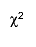$#chi^{2}$ test for comparing weighted and unweighted histograms

Function: Returns p-value. Other return values are specified by the 3rd parameter <br>

Parameters:

- h2: the second histogram
- option:
o "UU" = experiment experiment comparison (unweighted-unweighted)
o "UW" = experiment MC comparison (unweighted-weighted). Note that
the first histogram should be unweighted
o "WW" = MC MC comparison (weighted-weighted)
o "NORM" = to be used when one or both of the histograms is scaled
but the histogram originally was unweighted
o by default underflows and overlows are not included:
* "OF" = overflows included
* "UF" = underflows included
o "P" = print chi2, ndf, p_value, igood
o "CHI2" = returns chi2 instead of p-value
o "CHI2/NDF" = returns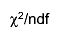$#chi^{2}/ndf$
- res: not empty - computes normalized residuals and returns them in
this array

The current implementation is based on the papers$#chi^{2}$ test for comparison
of weighted and unweighted histograms" in Proceedings of PHYSTAT05 and
"Comparison weighted and unweighted histograms", arXiv:physics/0605123
by N.Gagunashvili. This function has been implemented by Daniel Haertl in August 2006.

Introduction:

A frequently used technique in data analysis is the comparison of
histograms. First suggested by Pearson  the$#chi^{2}$ test of
homogeneity is used widely for comparing usual (unweighted) histograms.
This paper describes the implementation modified$#chi^{2}$ tests
for comparison of weighted and unweighted  histograms and two weighted
histograms  as well as usual Pearson's$#chi^{2}$ test for
comparison two usual (unweighted) histograms.

Overview:

Comparison of two histograms expect hypotheses that two histograms
represent the identical distributions. To make a decision p-value should
be calculated. The hypotheses of identity is rejected if p-value is
lower then some significance level. Traditionally significance levels
0.1, 0.05 and 0.01 are used. The comparison procedure should include an
analysis of the residuals which is often helpful in identifying the
bins of histograms responsible for a significant overall$#chi^{2}$ value.
Residuals are the difference between bin contents and expected bin
contents. Most convenient for analysis are the normalized residuals. If
hypotheses of identity are valid then normalized residuals are
approximately independent and identically distributed random variables
having N(0,1) distribution. Analysis of residuals expect test of above
mentioned properties of residuals. Notice that indirectly the analysis
of residuals increase the power of$#chi^{2}$ test.

Methods of comparison:$#chi^{2}$ test for comparison two (unweighted) histograms:
Let us consider two  histograms with the  same binning and the  number
of bins equal to r. Let us denote the number of events in the ith bin
in the first histogram as ni and as mi in the second one. The total
number of events in the first histogram is equal to: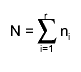$N = #sum_{i=1}^{r} n_{i}$
and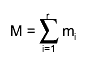$M = #sum_{i=1}^{r} m_{i}$
in the second histogram. The hypothesis of identity (homogeneity) 
is that the two histograms represent random values with identical
distributions. It is equivalent that there exist r constants p1,...,pr,
such that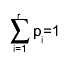$#sum_{i=1}^{r} p_{i}=1$
and the probability of belonging to the ith bin for some measured value
in both experiments is  equal to pi. The number of events in the ith
bin is a random variable with a distribution approximated by a Poisson
probability distribution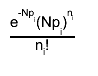$#frac{e^{-Np_{i}}(Np_{i})^{n_{i}}}{n_{i}!}$
for the first histogram and with distribution$#frac{e^{-Mp_{i}}(Mp_{i})^{m_{i}}}{m_{i}!}$
for the second histogram. If the hypothesis of homogeneity is valid,
then the  maximum likelihood estimator of pi, i=1,...,r, is$#hat{p}_{i}= #frac{n_{i}+m_{i}}{N+M}$
and then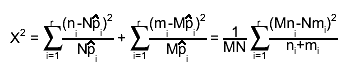$X^{2} = #sum_{i=1}^{r}#frac{(n_{i}-N#hat{p}_{i})^{2}}{N#hat{p}_{i}} + #sum_{i=1}^{r}#frac{(m_{i}-M#hat{p}_{i})^{2}}{M#hat{p}_{i}} = #frac{1}{MN} #sum_{i=1}^{r}#frac{(Mn_{i}-Nm_{i})^{2}}{n_{i}+m_{i}}$
has approximately a$#chi^{2}_{(r-1)}$ distribution .
The comparison procedure can include an analysis of the residuals which
is often helpful in identifying the bins of histograms responsible for
a significant overall$#chi^{2}$value. Most convenient for
analysis are the adjusted (normalized) residuals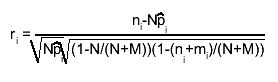$r_{i} = #frac{n_{i}-N#hat{p}_{i}}{#sqrt{N#hat{p}_{i}}#sqrt{(1-N/(N+M))(1-(n_{i}+m_{i})/(N+M))}}$
If hypotheses of  homogeneity are valid then residuals ri are
approximately independent and identically distributed random variables
having N(0,1) distribution. The application of the$#chi^{2}$ test has
restrictions related to the value of the expected frequencies Npi,
Mpi, i=1,...,r. A conservative rule formulated in  is that all the
expectations must be 1 or greater for both histograms. In practical
cases when expected frequencies are not known the estimated expected
frequencies$M#hat{p}_{i}, N#hat{p}_{i}, i=1,...,r$  can be used.

Unweighted and weighted histograms comparison:

A simple  modification of the  ideas described above can be used for the
comparison of the usual (unweighted) and weighted histograms. Let us
denote the number of events in the ith bin in the unweighted
histogram as ni and the common weight of events in the ith bin of the
weighted histogram as wi. The total number of events in the
unweighted histogram is equal to$N = #sum_{i=1}^{r} n_{i}$
and the total weight of events in the weighted histogram is equal to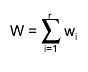$W = #sum_{i=1}^{r} w_{i}$
Let us formulate the hypothesis of identity of an unweighted histogram
to a weighted histogram so that there exist r constants p1,...,pr, such
that$#sum_{i=1}^{r} p_{i} = 1$
for the unweighted histogram. The weight wi is a random variable with a
distribution approximated by the normal probability distribution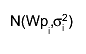$N(Wp_{i},#sigma_{i}^{2})$ where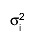$#sigma_{i}^{2}$ is the variance of the weight wi.
If we replace the variance$#sigma_{i}^{2}$
with estimate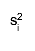$s_{i}^{2}$ (sum of squares of weights of
events in the ith bin) and the hypothesis of identity is valid, then the
maximum likelihood estimator of  pi,i=1,...,r, is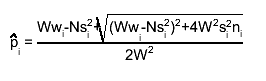$#hat{p}_{i} = #frac{Ww_{i}-Ns_{i}^{2}+#sqrt{(Ww_{i}-Ns_{i}^{2})^{2}+4W^{2}s_{i}^{2}n_{i}}}{2W^{2}}$
We may then use the test statistic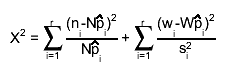$X^{2} = #sum_{i=1}^{r} #frac{(n_{i}-N#hat{p}_{i})^{2}}{N#hat{p}_{i}} + #sum_{i=1}^{r} #frac{(w_{i}-W#hat{p}_{i})^{2}}{s_{i}^{2}}$
and it has approximately a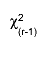$#chi^{2}_{(r-1)}$ distribution . This test, as well
as the original one , has a restriction on the expected frequencies. The
expected frequencies recommended for the weighted histogram is more than 25.
The value of the minimal expected frequency can be decreased down to 10 for
the case when the weights of the events are close to constant. In the case
of a weighted histogram if the number of events is unknown, then we can
apply this recommendation for the equivalent number of events as$n_{i}^{equiv} = #frac{ w_{i}^{2} }{ s_{i}^{2} }$
The minimal expected frequency for an unweighted histogram must be 1. Notice
that any usual (unweighted) histogram can be considered as a weighted
histogram with events that have constant weights equal to 1.
The variance$z_{i}^{2}$ of the difference between the weight wi
and the estimated expectation value of the weight is approximately equal to: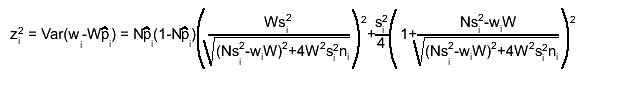$z_{i}^{2} = Var(w_{i}-W#hat{p}_{i}) = N#hat{p}_{i}(1-N#hat{p}_{i})#left(#frac{Ws_{i}^{2}}{#sqrt{(Ns_{i}^{2}-w_{i}W)^{2}+4W^{2}s_{i}^{2}n_{i}}}#right)^{2}+#frac{s_{i}^{2}}{4}#left(1+#frac{Ns_{i}^{2}-w_{i}W}{#sqrt{(Ns_{i}^{2}-w_{i}W)^{2}+4W^{2}s_{i}^{2}n_{i}}}#right)^{2}$
The  residuals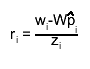$r_{i} = #frac{w_{i}-W#hat{p}_{i}}{z_{i}}$
have approximately a normal distribution with mean equal to 0 and standard
deviation  equal to 1.

Two weighted histograms comparison:

Let us denote the common  weight of events of the ith bin in the first
histogram as w1i and as w2i in the second one. The total weight of events
in the first histogram is equal to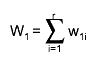$W_{1} = #sum_{i=1}^{r} w_{1i}$
and$W_{2} = #sum_{i=1}^{r} w_{2i}$
in the second histogram. Let us formulate the hypothesis of identity of
weighted histograms so that there exist r constants p1,...,pr, such that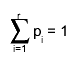$#sum_{i=1}^{r} p_{i} = 1$
and also expectation value of weight w1i equal to W1pi and expectation value
of weight w2i equal to W2pi. Weights in both the histograms are random
variables with distributions which can be approximated by a normal
probability distribution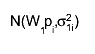$N(W_{1}p_{i},#sigma_{1i}^{2})$ for the first histogram
and by a distribution$N(W_{2}p_{i},#sigma_{2i}^{2})$ for the second.
Here$#sigma_{1i}^{2}$ and$#sigma_{2i}^{2}$ are the variances
of w1i and w2i with estimators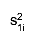$s_{1i}^{2}$ and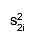$s_{2i}^{2}$ respectively.
If the hypothesis of identity is valid, then the maximum likelihood and
Least Square Method estimator of pi,i=1,...,r, is$#hat{p}_{i} = #frac{w_{1i}W_{1}/s_{1i}^{2}+w_{2i}W_{2} /s_{2i}^{2}}{W_{1}^{2}/s_{1i}^{2}+W_{2}^{2}/s_{2i}^{2}}$
We may then use the test statistic$X^{2} = #sum_{i=1}^{r} #frac{(w_{1i}-W_{1}#hat{p}_{i})^{2}}{s_{1i}^{2}} + #sum_{i=1}^{r} #frac{(w_{2i}-W_{2}#hat{p}_{i})^{2}}{s_{2i}^{2}} = #sum_{i=1}^{r} #frac{(W_{1}w_{2i}-W_{2}w_{1i})^{2}}{W_{1}^{2}s_{2i}^{2}+W_{2}^{2}s_{1i}^{2}}$
and it has approximately a$#chi^{2}_{(r-1)}$ distribution .
The normalized or studentised residuals$r_{i} = #frac{w_{1i}-W_{1}#hat{p}_{i}}{s_{1i}#sqrt{1 - #frac{1}{(1+W_{2}^{2}s_{1i}^{2}/W_{1}^{2}s_{2i}^{2})}}}$
have approximately a normal distribution with mean equal to 0 and standard
deviation 1. A recommended minimal expected frequency is equal to 10 for
the proposed test.

Numerical examples:

The method described herein is now illustrated with an example.
We take a distribution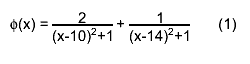$#phi(x) = #frac{2}{(x-10)^{2}+1} + #frac{1}{(x-14)^{2}+1} (1)$
defined on the interval [4,16]. Events distributed according to the formula
(1) are simulated to create the unweighted histogram. Uniformly distributed
events are simulated for the weighted histogram with weights calculated by
formula (1). Each histogram has the same number of bins: 20. Fig.1 shows
the result of comparison of the unweighted histogram with 200 events
(minimal expected frequency equal to one) and the weighted histogram with
500 events (minimal expected frequency equal to 25)Fig 1. An example of comparison of the unweighted histogram with 200 events
and the weighted histogram with 500 events:
a) unweighted histogram;
b) weighted histogram;
c) normalized residuals plot;
d) normal Q-Q plot of residuals.

The value of the test statistic$#chi^{2}$ is equal to
21.09 with p-value equal to 0.33, therefore the hypothesis of identity of
the two histograms can be accepted for 0.05 significant level. The behavior
of the normalized residuals plot (see Fig. 1c) and the normal Q-Q plot
(see Fig. 1d) of residuals are regular and we cannot identify the outliers
or bins with a big influence on$#chi^{2}$.

The second example presents the same two histograms but 17 events was added
to content of bin number 15 in unweighted histogram. Fig.2 shows the result
of comparison of the unweighted histogram with 217 events (minimal expected
frequency equal to one) and the weighted histogram with 500 events (minimal
expected frequency equal to 25)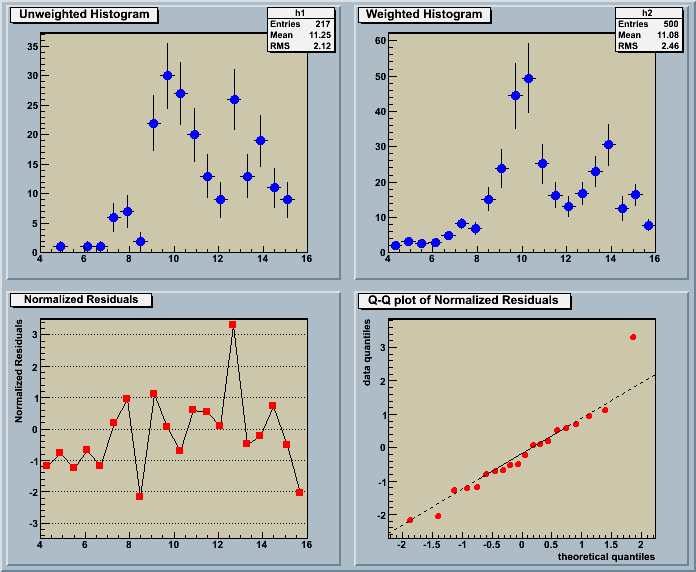Fig 2. An example of comparison of the unweighted histogram with 217 events
and the weighted histogram with 500 events:
a) unweighted histogram;
b) weighted histogram;
c) normalized residuals plot;
d) normal Q-Q plot of residuals.

The value of the test statistic$#chi^{2}$ is equal to
32.33 with p-value equal to 0.029, therefore the hypothesis of identity of
the two histograms is rejected for 0.05 significant level. The behavior of
the normalized residuals plot (see Fig. 2c) and the normal Q-Q plot (see
Fig. 2d) of residuals are not regular and we can identify the outlier or
bin with a big influence on$#chi^{2}$.

References:

 Pearson, K., 1904. On the Theory of Contingency and Its Relation to
Association and Normal Correlation. Drapers' Co. Memoirs, Biometric
Series No. 1, London.
 Gagunashvili, N., 2006.$#chi^{2}$ test for comparison
of weighted and unweighted histograms. Statistical Problems in Particle
Physics, Astrophysics and Cosmology, Proceedings of PHYSTAT05,
Oxford, UK, 12-15 September 2005, Imperial College Press, London, 43-44.
Gagunashvili,N., Comparison of weighted and unweighted histograms,
arXiv:physics/0605123, 2006.
 Cramer, H., 1946. Mathematical methods of statistics.
Princeton University Press, Princeton.
 Haberman, S.J., 1973. The analysis of residuals in cross-classified tables.
Biometrics 29, 205-220.
 Lewontin, R.C. and Felsenstein, J., 1965. The robustness of homogeneity
test in 2xN tables. Biometrics 21, 19-33.
 Seber, G.A.F., Lee, A.J., 2003, Linear Regression Analysis.
John Wiley & Sons Inc., New York.
```
Double_t Chi2TestX(const TH1* h2, Double_t& chi2, Int_t& ndf, Int_t& igood, Option_t* option = "UU", Double_t* res = 0) const
``` The computation routine of the Chisquare test. For the method description,
see Chi2Test() function.
Returns p-value
parameters:
- h2-second histogram
- option:
"UU" = experiment experiment comparison (unweighted-unweighted)
"UW" = experiment MC comparison (unweighted-weighted). Note that the first
histogram should be unweighted
"WW" = MC MC comparison (weighted-weighted)

"NORM" = if one or both histograms is scaled

"OF" = overflows included
"UF" = underflows included
by default underflows and overlows are not included

- igood:
igood=0 - no problems
For unweighted unweighted  comparison
igood=1'There is a bin in the 1st histogram with less than 1 event'
igood=2'There is a bin in the 2nd histogram with less than 1 event'
igood=3'when the conditions for igood=1 and igood=2 are satisfied'
For  unweighted weighted  comparison
igood=1'There is a bin in the 1st histogram with less then 1 event'
igood=2'There is a bin in the 2nd histogram with less then 10 effective number of events'
igood=3'when the conditions for igood=1 and igood=2 are satisfied'
For  weighted weighted  comparison
igood=1'There is a bin in the 1st  histogram with less then 10 effective
number of events'
igood=2'There is a bin in the 2nd  histogram with less then 10 effective
number of events'
igood=3'when the conditions for igood=1 and igood=2 are satisfied'

- chi2 - chisquare of the test
- ndf  - number of degrees of freedom (important, when both histograms have the same
empty bins)
- res -  normalized residuals for further analysis
```

```  Compute integral (cumulative sum of bins)
The result stored in fIntegral is used by the GetRandom functions.
This function is automatically called by GetRandom when the fIntegral
array does not exist or when the number of entries in the histogram
has changed since the previous call to GetRandom.
The resulting integral is normalized to 1
```

```  Return a pointer to the array of bins integral.
if the pointer fIntegral is null, TH1::ComputeIntegral is called
```
void Copy(TObject& hnew) const
```   -*-*-*-*-*Copy this histogram structure to newth1*-*-*-*-*-*-*-*-*-*-*-*

Note that this function does not copy the list of associated functions.
Use TObJect::Clone to make a full copy of an histogram.
```
void DirectoryAutoAdd(TDirectory* )
``` Perform the automatic addition of the histogram to the given directory

Note this function is called in place when the semantic requires
this object to be added to a directory (I.e. when being read from
a TKey or being Cloned)

```

```   -*-*-*-*-*-*-*-*-*Compute distance from point px,py to a line*-*-*-*-*-*

Compute the closest distance of approach from point px,py to elements
of an histogram.
The distance is computed in pixels units.

Algorithm:
Currently, this simple model computes the distance from the mouse
to the histogram contour only.

-*-*-*-*-*-*-*-*-*-*-*-*-*-*-*-*-*-*-*-*-*-*-*-*-*-*-*-*-*-*-*-*-*-*-*
```
void Divide(TF1* f1, Double_t c1 = 1)
``` Performs the operation: this = this/(c1*f1)
if errors are defined (see TH1::Sumw2), errors are also recalculated.

Only bins inside the function range are recomputed.
IMPORTANT NOTE: If you intend to use the errors of this histogram later
you should call Sumw2 before making this operation.
This is particularly important if you fit the histogram after TH1::Divide
```
void Divide(const TH1* h1)
```   -*-*-*-*-*-*-*-*-*Divide this histogram by h1*-*-*-*-*-*-*-*-*-*-*-*-*

this = this/h1
if errors are defined (see TH1::Sumw2), errors are also recalculated.
Note that if h1 has Sumw2 set, Sumw2 is automatically called for this
if not already set.
The resulting errors are calculated assuming uncorrelated histograms.
See the other TH1::Divide that gives the possibility to optionaly
compute Binomial errors.

IMPORTANT NOTE: If you intend to use the errors of this histogram later
you should call Sumw2 before making this operation.
This is particularly important if you fit the histogram after TH1::Scale
```
void Divide(const TH1* h1, const TH1* h2, Double_t c1 = 1, Double_t c2 = 1, Option_t* option = "")
```   -*-*-*Replace contents of this histogram by the division of h1 by h2*-*-*

this = c1*h1/(c2*h2)

if errors are defined (see TH1::Sumw2), errors are also recalculated
Note that if h1 or h2 have Sumw2 set, Sumw2 is automatically called for this
if not already set.
The resulting errors are calculated assuming uncorrelated histograms.
However, if option ="B" is specified, Binomial errors are computed.
In this case c1 and c2 do not make real sense and they are ignored.

IMPORTANT NOTE: If you intend to use the errors of this histogram later
you should call Sumw2 before making this operation.
This is particularly important if you fit the histogram after TH1::Divide

Please note also that in the binomial case errors are calculated using standard
binomial statistics, which means when b1 = b2, the error is zero.
If you prefer to have efficiency errors not going to zero when the efficiency is 1, you must
use the function TGraphAsymmErrors::BayesDivide, which will return an asymmetric and non-zero lower
error for the case b1=b2.
```
void Draw(Option_t* option = "")
```   -*-*-*-*-*-*-*-*-*Draw this histogram with options*-*-*-*-*-*-*-*-*-*-*-*

Histograms are drawn via the THistPainter class. Each histogram has
a pointer to its own painter (to be usable in a multithreaded program).
The same histogram can be drawn with different options in different pads.
When an histogram drawn in a pad is deleted, the histogram is
automatically removed from the pad or pads where it was drawn.
If an histogram is drawn in a pad, then filled again, the new status
of the histogram will be automatically shown in the pad next time
the pad is updated. One does not need to redraw the histogram.
To draw the current version of an histogram in a pad, one can use
h->DrawCopy();
This makes a clone of the histogram. Once the clone is drawn, the original
histogram may be modified or deleted without affecting the aspect of the
clone.
By default, TH1::Draw clears the current pad.

One can use TH1::SetMaximum and TH1::SetMinimum to force a particular
value for the maximum or the minimum scale on the plot.

TH1::UseCurrentStyle can be used to change all histogram graphics
attributes to correspond to the current selected style.
This function must be called for each histogram.
In case one reads and draws many histograms from a file, one can force
the histograms to inherit automatically the current graphics style
by calling before gROOT->ForceStyle();

See THistPainter::Paint for a description of all the drawing options

-*-*-*-*-*-*-*-*-*-*-*-*-*-*-*-*-*-*-*-*-*-*-*-*-*-*-*-*-*-*-*-*-*-*-*-*
```
TH1 * DrawCopy(Option_t* option = "") const
```   -*-*-*-*-*Copy this histogram and Draw in the current pad*-*-*-*-*-*-*-*

Once the histogram is drawn into the pad, any further modification
using graphics input will be made on the copy of the histogram,
and not to the original object.

See Draw for the list of options

-*-*-*-*-*-*-*-*-*-*-*-*-*-*-*-*-*-*-*-*-*-*-*-*-*-*-*-*-*-*-*-*-*-*-*-*
```
TH1 * DrawNormalized(Option_t* option = "", Double_t norm = 1) const
```  Draw a normalized copy of this histogram.

A clone of this histogram is normalized to norm and drawn with option.
A pointer to the normalized histogram is returned.
The contents of the histogram copy are scaled such that the new
sum of weights (excluding under and overflow) is equal to norm.
Note that the returned normalized histogram is not added to the list
of histograms in the current directory in memory.
It is the user's responsability to delete this histogram.
The kCanDelete bit is set for the returned object. If a pad containing
this copy is cleared, the histogram will be automatically deleted.
See also remark about calling Sumw2 before scaling a histogram to get
a correct computation of the error bars.

See Draw for the list of options

-*-*-*-*-*-*-*-*-*-*-*-*-*-*-*-*-*-*-*-*-*-*-*-*-*-*-*-*-*-*-*-*-*-*-*-*
```
void DrawPanel()
```   -*-*-*-*-*Display a panel with all histogram drawing options*-*-*-*-*-*

See class TDrawPanelHist for example
```
void Eval(TF1* f1, Option_t* option = "")
```   -*-*-*Evaluate function f1 at the center of bins of this histogram-*-*-*-*

If option "R" is specified, the function is evaluated only
for the bins included in the function range.
If option "A" is specified, the value of the function is added to the
existing bin contents
If option "S" is specified, the value of the function is used to
generate a value, distributed according to the Poisson
distribution, with f1 as the mean.

-*-*-*-*-*-*-*-*-*-*-*-*-*-*-*-*-*-*-*-*-*-*-*-*-*-*-*-*-*-*-*-*-*-*-*-*
```
void ExecuteEvent(Int_t event, Int_t px, Int_t py)
```   -*-*-*-*-*-*-*-*-*Execute action corresponding to one event*-*-*-*

This member function is called when a histogram is clicked with the locator

If Left button clicked on the bin top value, then the content of this bin
is modified according to the new position of the mouse when it is released.

-*-*-*-*-*-*-*-*-*-*-*-*-*-*-*-*-*-*-*-*-*-*-*-*-*-*-*-*-*-*-*-*-*-*-*
```
TH1* FFT(TH1* h_output, Option_t* option)
``` This function allows to do discrete Fourier transforms of TH1 and TH2.
Available transform types and flags are described below.

To extract more information about the transform, use the function
TVirtualFFT::GetCurrentTransform() to get a pointer to the current
transform object.

Parameters:
1st - histogram for the output. If a null pointer is passed, a new histogram is created
and returned, otherwise, the provided histogram is used and should be big enough

Options: option parameters consists of 3 parts:
- option on what to return
"RE" - returns a histogram of the real part of the output
"IM" - returns a histogram of the imaginary part of the output
"MAG"- returns a histogram of the magnitude of the output
"PH" - returns a histogram of the phase of the output

- option of transform type
"R2C"  - real to complex transforms - default
"R2HC" - real to halfcomplex (special format of storing output data,
results the same as for R2C)
"DHT" - discrete Hartley transform
real to real transforms (sine and cosine):
"R2R_0", "R2R_1", "R2R_2", "R2R_3" - discrete cosine transforms of types I-IV
"R2R_4", "R2R_5", "R2R_6", "R2R_7" - discrete sine transforms of types I-IV
To specify the type of each dimension of a 2-dimensional real to real
transform, use options of form "R2R_XX", for example, "R2R_02" for a transform,
which is of type "R2R_0" in 1st dimension and  "R2R_2" in the 2nd.

- option of transform flag
"ES" (from "estimate") - no time in preparing the transform, but probably sub-optimal
performance
"M" (from "measure")   - some time spend in finding the optimal way to do the transform
"P" (from "patient")   - more time spend in finding the optimal way to do the transform
"EX" (from "exhaustive") - the most optimal way is found
This option should be chosen depending on how many transforms of the same size and
type are going to be done. Planning is only done once, for the first transform of this
size and type. Default is "ES".
Examples of valid options: "Mag R2C M" "Re R2R_11" "Im R2C ES" "PH R2HC EX"
```

```   -*-*-*-*-*-*-*-*Increment bin with abscissa X by 1*-*-*-*-*-*-*-*-*-*-*

if x is less than the low-edge of the first bin, the Underflow bin is incremented
if x is greater than the upper edge of last bin, the Overflow bin is incremented

If the storage of the sum of squares of weights has been triggered,
via the function Sumw2, then the sum of the squares of weights is incremented
by 1 in the bin corresponding to x.

-*-*-*-*-*-*-*-*-*-*-*-*-*-*-*-*-*-*-*-*-*-*-*-*-*-*-*-*-*-*-*-*-*-*-*-*
```

```   -*-*-*-*-*-*Increment bin with abscissa X with a weight w*-*-*-*-*-*-*-*

if x is less than the low-edge of the first bin, the Underflow bin is incremented
if x is greater than the upper edge of last bin, the Overflow bin is incremented

If the storage of the sum of squares of weights has been triggered,
via the function Sumw2, then the sum of the squares of weights is incremented
by w^2 in the bin corresponding to x.

-*-*-*-*-*-*-*-*-*-*-*-*-*-*-*-*-*-*-*-*-*-*-*-*-*-*-*-*-*-*-*-*-*-*-*-*
```
Int_t Fill(const char *namex, Double_t w)
``` Increment bin with namex with a weight w

if x is less than the low-edge of the first bin, the Underflow bin is incremented
if x is greater than the upper edge of last bin, the Overflow bin is incremented

If the storage of the sum of squares of weights has been triggered,
via the function Sumw2, then the sum of the squares of weights is incremented
by w^2 in the bin corresponding to x.

```
void FillN(Int_t ntimes, const Double_t* x, const Double_t* w, Int_t stride = 1)
```   -*-*-*-*-*-*Fill this histogram with an array x and weights w*-*-*-*-*

ntimes:  number of entries in arrays x and w (array size must be ntimes*stride)
x:       array of values to be histogrammed
w:       array of weighs
stride:  step size through arrays x and w

If the storage of the sum of squares of weights has been triggered,
via the function Sumw2, then the sum of the squares of weights is incremented
by w[i]^2 in the bin corresponding to x[i].
if w is NULL each entry is assumed a weight=1

-*-*-*-*-*-*-*-*-*-*-*-*-*-*-*-*-*-*-*-*-*-*-*-*-*-*-*-*-*-*-*-*-*-*-*-*
```
void FillRandom(const char *fname, Int_t ntimes)
```   -*-*-*-*-*Fill histogram following distribution in function fname*-*-*-*

The distribution contained in the function fname (TF1) is integrated
over the channel contents.
It is normalized to 1.
Getting one random number implies:
- Generating a random number between 0 and 1 (say r1)
- Look in which bin in the normalized integral r1 corresponds to
- Fill histogram channel
ntimes random numbers are generated

One can also call TF1::GetRandom to get a random variate from a function.

-*-*-*-*-*-*-*-*-*-*-*-*-*-*-*-*-*-*-*-*-*-*-*-*-*-*-*-**-*-*-*-*-*-*-*
```
void FillRandom(TH1 *h, Int_t ntimes)
```   -*-*-*-*-*Fill histogram following distribution in histogram h*-*-*-*

The distribution contained in the histogram h (TH1) is integrated
over the channel contents.
It is normalized to 1.
Getting one random number implies:
- Generating a random number between 0 and 1 (say r1)
- Look in which bin in the normalized integral r1 corresponds to
- Fill histogram channel
ntimes random numbers are generated

-*-*-*-*-*-*-*-*-*-*-*-*-*-*-*-*-*-*-*-*-*-*-*-*-*-*-*-**-*-*-*-*-*-*-*
```
Int_t FindBin(Double_t x, Double_t y = 0, Double_t z = 0)
```   -*-*-*-*Return Global bin number corresponding to x,y,z*-*-*-*-*-*-*

2-D and 3-D histograms are represented with a one dimensional
structure.
This has the advantage that all existing functions, such as
GetBinContent, GetBinError, GetBinFunction work for all dimensions.
See also TH1::GetBin
-*-*-*-*-*-*-*-*-*-*-*-*-*-*-*-*-*-*-*-*-*-*-*-*-*-*-*-*-*-*-*-*-*-*-*
```
TObject * FindObject(const char *name)
``` search object named name in the list of functions
```
TObject * FindObject(const TObject *obj)
``` search object obj in the list of functions
```
Int_t Fit(const char *fname ,Option_t *option ,Option_t *goption, Double_t xxmin, Double_t xxmax)
```                     Fit histogram with function fname

fname is the name of an already predefined function created by TF1 or TF2
Predefined functions such as gaus, expo and poln are automatically
created by ROOT.
fname can also be a formula, accepted by the linear fitter (linear parts divided
by "++" sign), for example "x++sin(x)" for fitting "*x+*sin(x)"

This function finds a pointer to the TF1 object with name fname
and calls TH1::Fit(TF1 *f1,...)
```
Int_t Fit(TF1 *f1 ,Option_t *option ,Option_t *goption, Double_t xxmin, Double_t xxmax)
```                     Fit histogram with function f1

Fit this histogram with function f1.

The list of fit options is given in parameter option.
option = "W"  Set all weights to 1 for non empty bins; ignore error bars
= "WW" Set all weights to 1 including empty bins; ignore error bars
= "I"  Use integral of function in bin instead of value at bin center
= "L"  Use Loglikelihood method (default is chisquare method)
= "LL" Use Loglikelihood method and bin contents are not integers)
= "U"  Use a User specified fitting algorithm (via SetFCN)
= "Q"  Quiet mode (minimum printing)
= "V"  Verbose mode (default is between Q and V)
= "E"  Perform better Errors estimation using Minos technique
= "B"  Use this option when you want to fix one or more parameters
and the fitting function is like "gaus","expo","poln","landau".
= "M"  More. Improve fit results
= "R"  Use the Range specified in the function range
= "N"  Do not store the graphics function, do not draw
= "0"  Do not plot the result of the fit. By default the fitted function
is drawn unless the option"N" above is specified.
= "+"  Add this new fitted function to the list of fitted functions
(by default, any previous function is deleted)
= "C"  In case of linear fitting, don't calculate the chisquare
(saves time)
= "F"  If fitting a polN, switch to minuit fitter

When the fit is drawn (by default), the parameter goption may be used
to specify a list of graphics options. See TH1::Draw for a complete
list of these options.

In order to use the Range option, one must first create a function
with the expression to be fitted. For example, if your histogram
has a defined range between -4 and 4 and you want to fit a gaussian
only in the interval 1 to 3, you can do:
TF1 *f1 = new TF1("f1","gaus",1,3);
histo->Fit("f1","R");

Setting initial conditions

Parameters must be initialized before invoking the Fit function.
The setting of the parameter initial values is automatic for the
predefined functions : poln, expo, gaus, landau. One can however disable
this automatic computation by specifying the option "B".
Note that if a predefined function is defined with an argument,
eg, gaus(0), expo(1), you must specify the initial values for
the parameters.
You can specify boundary limits for some or all parameters via
f1->SetParLimits(p_number, parmin, parmax);
if parmin>=parmax, the parameter is fixed
Note that you are not forced to fix the limits for all parameters.
For example, if you fit a function with 6 parameters, you can do:
func->SetParameters(0,3.1,1.e-6,-8,0,100);
func->SetParLimits(3,-10,-4);
func->FixParameter(4,0);
func->SetParLimits(5, 1,1);
With this setup, parameters 0->2 can vary freely
Parameter 3 has boundaries [-10,-4] with initial value -8
Parameter 4 is fixed to 0
Parameter 5 is fixed to 100.
When the lower limit and upper limit are equal, the parameter is fixed.
However to fix a parameter to 0, one must call the FixParameter function.

Note that option "I" gives better results but is slower.

Changing the fitting function

By default the fitting function H1FitChisquare is used.
To specify a User defined fitting function, specify option "U" and
call the following functions:
TVirtualFitter::Fitter(myhist)->SetFCN(MyFittingFunction)
where MyFittingFunction is of type:
extern void MyFittingFunction(Int_t &npar, Double_t *gin, Double_t &f, Double_t *u, Int_t flag);

Fitting a histogram of dimension N with a function of dimension N-1

It is possible to fit a TH2 with a TF1 or a TH3 with a TF2.
In this case the option "Integral" is not allowed and each cell has
equal weight.

Associated functions

One or more object (typically a TF1*) can be added to the list
of functions (fFunctions) associated to each histogram.
When TH1::Fit is invoked, the fitted function is added to this list.
Given an histogram h, one can retrieve an associated function
with:  TF1 *myfunc = h->GetFunction("myfunc");

Access to the fit status

The function return the status of the fit (fitResult) in the following form
fitResult = migradResult + 10*minosResult + 100*hesseResult + 1000*improveResult
The fitResult is 0 is the fit is OK.
The fitResult is negative in case of an error not connected with the fit.

Access to the fit results

If the histogram is made persistent, the list of
associated functions is also persistent. Given a pointer (see above)
to an associated function myfunc, one can retrieve the function/fit
parameters with calls such as:
Double_t chi2 = myfunc->GetChisquare();
Double_t par0 = myfunc->GetParameter(0); //value of 1st parameter
Double_t err0 = myfunc->GetParError(0);  //error on first parameter

Access to the fit covariance matrix

Example1:
TH1F h("h","test",100,-2,2);
h.FillRandom("gaus",1000);
h.Fit("gaus");
Double_t matrix;
gMinuit->mnemat(&matrix,3);
Example2:
TH1F h("h","test",100,-2,2);
h.FillRandom("gaus",1000);
h.Fit("gaus");
TVirtualFitter *fitter = TVirtualFitter::GetFitter();
TMatrixD matrix(npar,npar,fitter->GetCovarianceMatrix());
Double_t errorFirstPar = fitter->GetCovarianceMatrixElement(0,0);

Changing the maximum number of parameters

By default, the fitter TMinuit is initialized with a maximum of 25 parameters.
You can redefine this default value by calling :
TVirtualFitter::Fitter(0,150); //to get a maximum of 150 parameters

Excluding points

Use TF1::RejectPoint inside your fitting function to exclude points
within a certain range from the fit. Example:
Double_t fline(Double_t *x, Double_t *par)
{
if (x > 2.5 && x < 3.5) {
TF1::RejectPoint();
return 0;
}
return par + par*x;
}

void exclude() {
TF1 *f1 = new TF1("f1"," +*x +gaus(2)",0,5);
f1->SetParameters(6,-1,5,3,0.2);
TH1F *h = new TH1F("h","background + signal",100,0,5);
h->FillRandom("f1",2000);
TF1 *fline = new TF1("fline",fline,0,5,2);
fline->SetParameters(2,-1);
h->Fit("fline","l");
}

Warning when using the option "0"

When selecting the option "0", the fitted function is added to
the list of functions of the histogram, but it is not drawn.
You can undo what you disabled in the following way:
h.Fit("myFunction","0"); // fit, store function but do not draw
h.Draw(); function is not drawn
const Int_t kNotDraw = 1<<9;
h.GetFunction("myFunction")->ResetBit(kNotDraw);
h.Draw();  // function is visible again

Access to the Fitter information during fitting

This function calls only the abstract fitter TVirtualFitter.
The default fitter is TFitter (calls TMinuit).
The default fitter can be set in the resource file in etc/system.rootrc
Root.Fitter:      Fumili
A different fitter can also be set via TVirtualFitter::SetDefaultFitter.
For example, to call the "Fumili" fitter instead of "Minuit", do
TVirtualFitter::SetDefaultFitter("Fumili");
During the fitting process, the objective function:
chisquare, likelihood or any user defined algorithm
is called (see eg in the TFitter class, the static functions
H1FitChisquare, H1FitLikelihood).
This objective function, in turn, calls the user theoretical function.
This user function is a static function called from the TF1 *f1 function.
Inside this user defined theoretical function , one can access:
TVirtualFitter *fitter = TVirtualFitter::GetFitter();  //the current fitter
TH1 *hist = (TH1*)fitter->GetObjectFit(); //the histogram being fitted
TF1 +f1 = (TF1*)fitter->GetUserFunction(); //the user theoretical function

By default, the fitter TMinuit is initialized with a maximum of 25 parameters.
For fitting linear functions (containing the "++" sign" and polN functions,
the linear fitter is initialized.

-*-*-*-*-*-*-*-*-*-*-*-*-*-*-*-*-*-*-*-*-*-*-*-*-*-*-*-*-*-*-*-*-*-*-*-*
```
void FitPanel()
```   -*-*-*-*-*Display a panel with all histogram fit options*-*-*-*-*-*

See class TFitPanel for example
```
TH1 * GetAsymmetry(TH1* h2, Double_t c2 = 1, Double_t dc2 = 0)
```  return an histogram containing the asymmetry of this histogram with h2,
where the asymmetry is defined as:

Asymmetry = (h1 - h2)/(h1 + h2)  where h1 = this

works for 1D, 2D, etc. histograms
c2 is an optional argument that gives a relative weight between the two
histograms, and dc2 is the error on this weight.  This is useful, for example,
when forming an asymmetry between two histograms from 2 different data sets that
need to be normalized to each other in some way.  The function calculates
the errors asumming Poisson statistics on h1 and h2 (that is, dh = sqrt(h)).

example:  assuming 'h1' and 'h2' are already filled

h3 = h1->GetAsymmetry(h2)

then 'h3' is created and filled with the asymmetry between 'h1' and 'h2';
h1 and h2 are left intact.

Note that it is the user's responsibility to manage the created histogram.

code proposed by Jason Seely (seely@mit.edu) and adapted by R.Brun

clone the histograms so top and bottom will have the
correct dimensions:
Sumw2 just makes sure the errors will be computed properly
when we form sums and ratios below.
```

``` static function
return the default buffer size for automatic histograms
the parameter fgBufferSize may be changed via SetDefaultBufferSize
```

``` static function
return kTRUE if TH1::Sumw2 must be called when creating new histograms.
see TH1::SetDefaultSumw2.
```

``` return the current number of entries
```

``` number of effective entries of the histogram,
i.e. the number of unweighted entries a histogram would need to
have the same statistical power as this histogram with possibly
weighted entries (i.e. <= TH1::GetEntries())
```
char * GetObjectInfo(Int_t px, Int_t py) const
```   Redefines TObject::GetObjectInfo.
Displays the histogram info (bin number, contents, integral up to bin
corresponding to cursor position px,py

```
TVirtualHistPainter * GetPainter(Option_t* option = "")
``` return pointer to painter
if painter does not exist, it is created
```
Int_t GetQuantiles(Int_t nprobSum, Double_t* q, const Double_t* probSum = 0)
```  Compute Quantiles for this histogram
Quantile x_q of a probability distribution Function F is defined as

F(x_q) = q with 0 <= q <= 1.

For instance the median x_0.5 of a distribution is defined as that value
of the random variable for which the distribution function equals 0.5:

F(x_0.5) = Probability(x < x_0.5) = 0.5

code from Eddy Offermann, Renaissance

input parameters
- this 1-d histogram (TH1F,D,etc). Could also be a TProfile
- nprobSum maximum size of array q and size of array probSum (if given)
- probSum array of positions where quantiles will be computed.
if probSum is null, probSum will be computed internally and will
have a size = number of bins + 1 in h. it will correspond to the
quantiles calculated at the lowest edge of the histogram (quantile=0) and
all the upper edges of the bins.
if probSum is not null, it is assumed to contain at least nprobSum values.
output
- return value nq (<=nprobSum) with the number of quantiles computed
- array q filled with nq quantiles

Note that the Integral of the histogram is automatically recomputed
if the number of entries is different of the number of entries when
the integral was computed last time. In case you do not use the Fill
functions to fill your histogram, but SetBinContent, you must call
TH1::ComputeIntegral before calling this function.

Getting quantiles q from two histograms and storing results in a TGraph,
a so-called QQ-plot

TGraph *gr = new TGraph(nprob);
h1->GetQuantiles(nprob,gr->GetX());
h2->GetQuantiles(nprob,gr->GetY());
gr->Draw("alp");

Example:
void quantiles() {
// demo for quantiles
const Int_t nq = 20;
TH1F *h = new TH1F("h","demo quantiles",100,-3,3);
h->FillRandom("gaus",5000);

Double_t xq[nq];  // position where to compute the quantiles in [0,1]
Double_t yq[nq];  // array to contain the quantiles
for (Int_t i=0;i<nq;i++) xq[i] = Float_t(i+1)/nq;
h->GetQuantiles(nq,yq,xq);

//show the original histogram in the top pad
TCanvas *c1 = new TCanvas("c1","demo quantiles",10,10,700,900);
c1->Divide(1,2);
c1->cd(1);
h->Draw();

// show the quantiles in the bottom pad
c1->cd(2);
gPad->SetGrid();
TGraph *gr = new TGraph(nq,xq,yq);
gr->SetMarkerStyle(21);
gr->Draw("alp");
}
```
Int_t FitOptionsMake(Option_t* option, Foption_t& Foption)
```   -*-*-*-*-*-*-*Decode string choptin and fill fitOption structure*-*-*-*-*-*

```
Int_t GetBin(Int_t binx, Int_t biny = 0, Int_t binz = 0) const
```   -*-*-*-*Return Global bin number corresponding to binx,y,z*-*-*-*-*-*-*

2-D and 3-D histograms are represented with a one dimensional
structure.
This has the advantage that all existing functions, such as
GetBinContent, GetBinError, GetBinFunction work for all dimensions.

In case of a TH1x, returns binx directly.
see TH1::GetBinXYZ for the inverse transformation.

Convention for numbering bins

For all histogram types: nbins, xlow, xup
bin = 0;       underflow bin
bin = 1;       first bin with low-edge xlow INCLUDED
bin = nbins;   last bin with upper-edge xup EXCLUDED
bin = nbins+1; overflow bin
In case of 2-D or 3-D histograms, a "global bin" number is defined.
For example, assuming a 3-D histogram with binx,biny,binz, the function
Int_t bin = h->GetBin(binx,biny,binz);
returns a global/linearized bin number. This global bin is useful
to access the bin information independently of the dimension.
-*-*-*-*-*-*-*-*-*-*-*-*-*-*-*-*-*-*-*-*-*-*-*-*-*-*-*-*-*-*-*-*-*-*-*
```
void GetBinXYZ(Int_t binglobal, Int_t& binx, Int_t& biny, Int_t& binz) const
``` return binx, biny, binz corresponding to the global bin number globalbin
see TH1::GetBin function above
```

``` return a random number distributed according the histogram bin contents.
This function checks if the bins integral exists. If not, the integral
is evaluated, normalized to one.
The integral is automatically recomputed if the number of entries
is not the same then when the integral was computed.
NB Only valid for 1-d histograms. Use GetRandom2 or 3 otherwise.
```
Double_t GetBinContent(Int_t bin) const
```   -*-*-*-*-*Return content of bin number bin

Implemented in TH1C,S,F,D

Convention for numbering bins

For all histogram types: nbins, xlow, xup
bin = 0;       underflow bin
bin = 1;       first bin with low-edge xlow INCLUDED
bin = nbins;   last bin with upper-edge xup EXCLUDED
bin = nbins+1; overflow bin
In case of 2-D or 3-D histograms, a "global bin" number is defined.
For example, assuming a 3-D histogram with binx,biny,binz, the function
Int_t bin = h->GetBin(binx,biny,binz);
returns a global/linearized bin number. This global bin is useful
to access the bin information independently of the dimension.
```
Double_t GetBinContent(Int_t binx, Int_t biny) const
```   -*-*-*-*-*Return content of bin number binx, biny

NB: Function to be called for 2-d histograms only
see convention for numbering bins in TH1::GetBin
```
Double_t GetBinContent(Int_t binx, Int_t biny, Int_t binz) const
```   -*-*-*-*-*Return content of bin number binx,biny,binz

NB: Function to be called for 3-d histograms only
see convention for numbering bins in TH1::GetBin
```
Double_t GetBinWithContent(Double_t c, Int_t& binx, Int_t firstx = 0, Int_t lastx = 0, Double_t maxdiff = 0) const
``` compute first binx in the range [firstx,lastx] for which
diff = abs(bin_content-c) <= maxdiff
In case several bins in the specified range with diff=0 are found
the first bin found is returned in binx.
In case several bins in the specified range satisfy diff <=maxdiff
the bin with the smallest difference is returned in binx.
In all cases the function returns the smallest difference.

NOTE1: if firstx <= 0, firstx is set to bin 1
if (lastx < firstx then firstx is set to the number of bins
ie if firstx=0 and lastx=0 (default) the search is on all bins.
NOTE2: if maxdiff=0 (default), the first bin with content=c is returned.
```

``` return a pointer to the X axis object
```

``` return a pointer to the Y axis object
```

``` return a pointer to the Z axis object
```

``` Given a point x, approximates the value via linear interpolation
based on the two nearest bin centers
Andy Mastbaum 10/21/08
```

void LabelsDeflate(Option_t* axis = "X")
``` Reduce the number of bins for this axis to the number of bins having a label.
```
void LabelsInflate(Option_t* axis = "X")
``` Double the number of bins for axis.
Refill histogram
This function is called by TAxis::FindBin(const char *label)
```
void LabelsOption(Option_t* option = "h", Option_t* axis = "X")
```  Set option(s) to draw axis with labels
option = "a" sort by alphabetic order
= ">" sort by decreasing values
= "<" sort by increasing values
= "h" draw labels horizonthal
= "v" draw labels vertical
= "u" draw labels up (end of label right adjusted)
= "d" draw labels down (start of label left adjusted)
```
Bool_t SameLimitsAndNBins(const TAxis& axis1, const TAxis& axis2)
``` Same limits and bins.
```
Bool_t RecomputeAxisLimits(TAxis& destAxis, const TAxis& anAxis)
``` Finds new limits for the axis for the Merge function.
returns false if the limits are incompatible
```
Long64_t Merge(TCollection* list)
``` Add all histograms in the collection to this histogram.
This function computes the min/max for the x axis,
compute a new number of bins, if necessary,
add bin contents, errors and statistics.
If all histograms have bin labels, bins with identical labels
will be merged, no matter what their order is.
If overflows are present and limits are different the function will fail.
The function returns the total number of entries in the result histogram
if the merge is successfull, -1 otherwise.

IMPORTANT remark. The axis x may have different number
of bins and different limits, BUT the largest bin width must be
a multiple of the smallest bin width and the upper limit must also
be a multiple of the bin width.
Example:
void atest() {
TH1F *h1 = new TH1F("h1","h1",110,-110,0);
TH1F *h2 = new TH1F("h2","h2",220,0,110);
TH1F *h3 = new TH1F("h3","h3",330,-55,55);
TRandom r;
for (Int_t i=0;i<10000;i++) {
h1->Fill(r.Gaus(-55,10));
h2->Fill(r.Gaus(55,10));
h3->Fill(r.Gaus(0,10));
}

TList *list = new TList;
list->Add(h1);
list->Add(h2);
list->Add(h3);
TH1F *h = (TH1F*)h1->Clone("h");
h->Reset();
h.Merge(list);
h->Draw();
}
```
void Multiply(TF1* h1, Double_t c1 = 1)
``` Performs the operation: this = this*c1*f1
if errors are defined (see TH1::Sumw2), errors are also recalculated.

Only bins inside the function range are recomputed.
IMPORTANT NOTE: If you intend to use the errors of this histogram later
you should call Sumw2 before making this operation.
This is particularly important if you fit the histogram after TH1::Multiply
```
void Multiply(const TH1* h1)
```   -*-*-*-*-*-*-*-*-*Multiply this histogram by h1*-*-*-*-*-*-*-*-*-*-*-*-*

this = this*h1

If errors of this are available (TH1::Sumw2), errors are recalculated.
Note that if h1 has Sumw2 set, Sumw2 is automatically called for this
if not already set.

IMPORTANT NOTE: If you intend to use the errors of this histogram later
you should call Sumw2 before making this operation.
This is particularly important if you fit the histogram after TH1::Multiply
```
void Multiply(const TH1* h1, const TH1* h2, Double_t c1 = 1, Double_t c2 = 1, Option_t* option = "")
```   -*-*-*Replace contents of this histogram by multiplication of h1 by h2*-*

this = (c1*h1)*(c2*h2)

If errors of this are available (TH1::Sumw2), errors are recalculated.
Note that if h1 or h2 have Sumw2 set, Sumw2 is automatically called for this
if not already set.

IMPORTANT NOTE: If you intend to use the errors of this histogram later
you should call Sumw2 before making this operation.
This is particularly important if you fit the histogram after TH1::Multiply
```
void Paint(Option_t* option = "")
```   -*-*-*-*-*-*-*Control routine to paint any kind of histograms*-*-*-*-*-*-*

This function is automatically called by TCanvas::Update.
(see TH1::Draw for the list of options)
```
TH1 * Rebin(Int_t ngroup = 2, const char* newname = "", const Double_t* xbins = 0)
```   Rebin this histogram

-case 1  xbins=0
if newname is not blank a new temporary histogram hnew is created.
else the current histogram is modified (default)
The parameter ngroup indicates how many bins of this have to me merged
into one bin of hnew
If the original histogram has errors stored (via Sumw2), the resulting
histograms has new errors correctly calculated.

examples: if h1 is an existing TH1F histogram with 100 bins
h1->Rebin();  //merges two bins in one in h1: previous contents of h1 are lost
h1->Rebin(5); //merges five bins in one in h1
TH1F *hnew = h1->Rebin(5,"hnew"); // creates a new histogram hnew
//merging 5 bins of h1 in one bin

NOTE:  If ngroup is not an exact divider of the number of bins,
the top limit of the rebinned histogram is changed
to the upper edge of the bin=newbins*ngroup and the corresponding
bins are added to the overflow bin.
Statistics will be recomputed from the new bin contents.

-case 2  xbins!=0
a new histogram is created (you should specify newname).
The parameter is the number of variable size bins in the created histogram.
The array xbins must contain ngroup+1 elements that represent the low-edge
of the bins.
If the original histogram has errors stored (via Sumw2), the resulting
histograms has new errors correctly calculated.

examples: if h1 is an existing TH1F histogram with 100 bins
Double_t xbins = {...} array of low-edges (xbins is the upper edge of last bin
h1->Rebin(24,"hnew",xbins);  //creates a new variable bin size histogram hnew
```
Bool_t FindNewAxisLimits(const TAxis* axis, const Double_t point, Double_t& newMin, Double_t& newMax)
``` finds new limits for the axis so that *point* is within the range and
the limits are compatible with the previous ones (see TH1::Merge).
new limits are put into *newMin* and *newMax* variables.
axis - axis whose limits are to be recomputed
point - point that should fit within the new axis limits
newMin - new minimum will be stored here
newMax - new maximum will be stored here.
false if failed (e.g. if the initial axis limits are wrong
or the new range is more than 2^64 times the old one).
```
void RebinAxis(Double_t x, TAxis* axis)
``` Histogram is resized along axis such that x is in the axis range.
The new axis limits are recomputed by doubling iteratively
the current axis range until the specified value x is within the limits.
The algorithm makes a copy of the histogram, then loops on all bins
of the old histogram to fill the rebinned histogram.
Takes into account errors (Sumw2) if any.
The algorithm works for 1-d, 2-d and 3-d histograms.
The bit kCanRebin must be set before invoking this function.
Ex:  h->SetBit(TH1::kCanRebin);
```
void RecursiveRemove(TObject* obj)
``` Recursively remove object from the list of functions
```
void Scale(Double_t c1 = 1, Option_t* option = "")
```   -*-*-*Multiply this histogram by a constant c1*-*-*-*-*-*-*-*-*

this = c1*this

Note that both contents and errors(if any) are scaled.
This function uses the services of TH1::Add

IMPORTANT NOTE: If you intend to use the errors of this histogram later
you should call Sumw2 before making this operation.
This is particularly important if you fit the histogram after TH1::Scale

One can scale an histogram such that the bins integral is equal to
the normalization parameter via TH1::Scale(Double_t norm), where norm
is the desired normalization divided by the integral of the histogram.

If option contains "width" the bin contents and errors are divided
by the bin width.
```
void SetDefaultBufferSize(Int_t buffersize = 1000)
``` static function to set the default buffer size for automatic histograms.
When an histogram is created with one of its axis lower limit greater
or equal to its upper limit, the function SetBuffer is automatically
called with the default buffer size.
```
void SetDefaultSumw2(Bool_t sumw2 = kTRUE)
``` static function.
When this static function is called with sumw2=kTRUE, all new
histograms will automatically activate the storage
of the sum of squares of errors, ie TH1::Sumw2 is automatically called.
```
void SetTitle(const char* title)
``` Change (i.e. set) the title

if title is in the form "stringt;stringx;stringy;stringz"
the histogram title is set to stringt, the x axis title to stringx,
the y axis title to stringy, and the z axis title to stringz.
To insert the character ";" in one of the titles, one should use "#;"
or "#semicolon".
```
void SmoothArray(Int_t NN, Double_t* XX, Int_t ntimes = 1)
``` smooth array xx, translation of Hbook routine hsmoof.F
based on algorithm 353QH twice presented by J. Friedman
in Proc.of the 1974 CERN School of Computing, Norway, 11-24 August, 1974.
```
void Smooth(Int_t ntimes = 1, Option_t* option = "")
``` Smooth bin contents of this histogram.
if option contains "R" smoothing is applied only to the bins
defined in the X axis range (default is to smooth all bins)
Bin contents are replaced by their smooth values.
Errors (if any) are not modified.
the smoothing procedure is repeated ntimes (default=1)
```
void StatOverflows(Bool_t flag = kTRUE)
```  if flag=kTRUE, underflows and overflows are used by the Fill functions
in the computation of statistics (mean value, RMS).
By default, underflows or overflows are not used.
```
void Streamer(TBuffer& b)
```   -*-*-*-*-*-*-*Stream a class object*-*-*-*-*-*-*-*-*-*-*-*-*-*-*-*

```
void Print(Option_t* option = "") const
```   -*-*-*-*-*Print some global quantities for this histogram*-*-*-*-*-*-*-*

If option "base" is given, number of bins and ranges are also printed
If option "range" is given, bin contents and errors are also printed
for all bins in the current range (default 1-->nbins)
If option "all" is given, bin contents and errors are also printed
for all bins including under and overflows.

```
void Rebuild(Option_t* option = "")
``` Using the current bin info, recompute the arrays for contents and errors
```
void Reset(Option_t* option = "")
```   -*-*-*-*-*-*Reset this histogram: contents, errors, etc*-*-*-*-*-*-*-*

if option "ICE" is specified, resets only Integral, Contents and Errors.
if option "M"   is specified, resets also Minimum and Maximum
```
void SavePrimitive(ostream& out, Option_t* option = "")
``` Save primitive as a C++ statement(s) on output stream out
```
void SavePrimitiveHelp(ostream& out, Option_t* option = "")
``` helper function for the SavePrimitive functions from TH1
or classes derived from TH1, eg TProfile, TProfile2D.
```
void UseCurrentStyle()
```   Copy current attributes from/to current style
```
Double_t GetMean(Int_t axis = 1) const
```  For axis = 1,2 or 3 returns the mean value of the histogram along
X,Y or Z axis.
For axis = 11, 12, 13 returns the standard error of the mean value
of the histogram along X, Y or Z axis

Note that the mean value/RMS is computed using the bins in the currently
defined range (see TAxis::SetRange). By default the range includes
all bins from 1 to nbins included, excluding underflows and overflows.
To force the underflows and overflows in the computation, one must
call the static function TH1::StatOverflows(kTRUE) before filling
the histogram.
```
Double_t GetMeanError(Int_t axis = 1) const
```   -*-*-*-*-*-*Return standard error of mean of this histogram along the X axis*-*-*-*-*

Note that the mean value/RMS is computed using the bins in the currently
defined range (see TAxis::SetRange). By default the range includes
all bins from 1 to nbins included, excluding underflows and overflows.
To force the underflows and overflows in the computation, one must
call the static function TH1::StatOverflows(kTRUE) before filling
the histogram.
Also note, that although the definition of standard error doesn't include the
assumption of normality, many uses of this feature implicitly assume it.
```
Double_t GetRMS(Int_t axis = 1) const
```  For axis = 1,2 or 3 returns the Sigma value of the histogram along
X, Y or Z axis
For axis = 11, 12 or 13 returns the error of RMS estimation along
X, Y or Z axis for Normal distribution

Note that the mean value/sigma is computed using the bins in the currently
defined range (see TAxis::SetRange). By default the range includes
all bins from 1 to nbins included, excluding underflows and overflows.
To force the underflows and overflows in the computation, one must
call the static function TH1::StatOverflows(kTRUE) before filling
the histogram.
Note that this function returns the Standard Deviation (Sigma)
of the distribution (not RMS).
The Sigma estimate is computed as Sqrt((1/N)*(Sum(x_i-x_mean)^2))
The name "RMS" was introduced many years ago (Hbook/PAW times).
We kept the name for continuity.
```
Double_t GetRMSError(Int_t axis = 1) const
```  Return error of RMS estimation for Normal distribution

Note that the mean value/RMS is computed using the bins in the currently
defined range (see TAxis::SetRange). By default the range includes
all bins from 1 to nbins included, excluding underflows and overflows.
To force the underflows and overflows in the computation, one must
call the static function TH1::StatOverflows(kTRUE) before filling
the histogram.
Value returned is standard deviation of sample standard deviation.
Note that it is an approximated value which is valid only in the case that the
original data distribution is Normal. The correct one would require
the 4-th momentum value, which cannot be accuratly estimated from an histogram since
the x-information for all entries is not kept.
```
Double_t GetSkewness(Int_t axis = 1) const
```For axis = 1, 2 or 3 returns skewness of the histogram along x, y or z axis.
For axis = 11, 12 or 13 returns the approximate standard error of skewness
of the histogram along x, y or z axis
Note, that since third and fourth moment are not calculated
at the fill time, skewness and its standard error are computed bin by bin
```
Double_t GetKurtosis(Int_t axis = 1) const
```For axis =1, 2 or 3 returns kurtosis of the histogram along x, y or z axis.
Kurtosis(gaussian(0, 1)) = 0.
For axis =11, 12 or 13 returns the approximate standard error of kurtosis
of the histogram along x, y or z axis
Note, that since third and fourth moment are not calculated
at the fill time, kurtosis and its standard error are computed bin by bin
```
void GetStats(Double_t* stats) const
``` fill the array stats from the contents of this histogram
The array stats must be correctly dimensionned in the calling program.
stats = sumw
stats = sumw2
stats = sumwx
stats = sumwx2

If no axis-subrange is specified (via TAxis::SetRange), the array stats
is simply a copy of the statistics quantities computed at filling time.
If a sub-range is specified, the function recomputes these quantities
from the bin contents in the current axis range.

Note that the mean value/RMS is computed using the bins in the currently
defined range (see TAxis::SetRange). By default the range includes
all bins from 1 to nbins included, excluding underflows and overflows.
To force the underflows and overflows in the computation, one must
call the static function TH1::StatOverflows(kTRUE) before filling
the histogram.
```
void PutStats(Double_t* stats)
``` Replace current statistics with the values in array stats
```

```   -*-*-*-*-*-*Return the sum of weights excluding under/overflows*-*-*-*-*

```
Double_t Integral(Option_t* option = "") const
```Return integral of bin contents. Only bins in the bins range are considered.
By default the integral is computed as the sum of bin contents in the range.
if option "width" is specified, the integral is the sum of
the bin contents multiplied by the bin width in x.
```
Double_t Integral(Int_t binx1, Int_t binx2, Option_t* option = "") const
```Return integral of bin contents between binx1 and binx2 for a 1-D histogram
By default the integral is computed as the sum of bin contents in the range.
if option "width" is specified, the integral is the sum of
the bin contents multiplied by the bin width in x.
```
Double_t KolmogorovTest(const TH1* h2, Option_t* option = "") const
```  Statistical test of compatibility in shape between
THIS histogram and h2, using Kolmogorov test.

Default: Ignore under- and overflow bins in comparison

option is a character string to specify options
"U" include Underflows in test  (also for 2-dim)
"O" include Overflows     (also valid for 2-dim)
"N" include comparison of normalizations
"D" Put out a line of "Debug" printout
"M" Return the Maximum Kolmogorov distance instead of prob
"X" Run the pseudo experiments post-processor with the following procedure:
make pseudoexperiments based on random values from the parent
distribution and compare the KS distance of the pseudoexperiment
to the parent distribution. Bin the KS distances in a histogram,
and then take the integral of all the KS values above the value
obtained from the original data to Monte Carlo distribution.
The number of pseudo-experiments nEXPT is currently fixed at 1000.
The function returns the integral.
(thanks to Ben Kilminster to submit this procedure). Note that
this option "X" is much slower.

The returned function value is the probability of test
(much less than one means NOT compatible)

Code adapted by Rene Brun from original HBOOK routine HDIFF

NOTE1
A good description of the Kolmogorov test can be seen at:
http://www.itl.nist.gov/div898/handbook/eda/section3/eda35g.htm

NOTE2
see also alternative function TH1::Chi2Test
The Kolmogorov test is assumed to give better results than Chi2Test
in case of histograms with low statistics.

NOTE3 (Jan Conrad, Fred James)
"The returned value PROB is calculated such that it will be
uniformly distributed between zero and one for compatible histograms,
provided the data are not binned (or the number of bins is very large
compared with the number of events). Users who have access to unbinned
data and wish exact confidence levels should therefore not put their data
into histograms, but should call directly TMath::KolmogorovTest. On
the other hand, since TH1 is a convenient way of collecting data and
saving space, this function has been provided. However, the values of
PROB for binned data will be shifted slightly higher than expected,
depending on the effects of the binning. For example, when comparing two
uniform distributions of 500 events in 100 bins, the values of PROB,
instead of being exactly uniformly distributed between zero and one, have
a mean value of about 0.56. We can apply a useful
rule: As long as the bin width is small compared with any significant
physical effect (for example the experimental resolution) then the binning
cannot have an important effect. Therefore, we believe that for all
practical purposes, the probability value PROB is calculated correctly
provided the user is aware that:
1. The value of PROB should not be expected to have exactly the correct
distribution for binned data.
2. The user is responsible for seeing to it that the bin widths are
small compared with any physical phenomena of interest.
3. The effect of binning (if any) is always to make the value of PROB
slightly too big. That is, setting an acceptance criterion of (PROB>0.05
will assure that at most 5% of truly compatible histograms are rejected,
and usually somewhat less."
```
void SetContent(const Double_t* content)
```   -*-*-*-*-*-*Replace bin contents by the contents of array content*-*-*-*

```
Int_t GetContour(Double_t* levels = 0)
```  Return contour values into array levels if pointer levels is non zero

The function returns the number of contour levels.
see GetContourLevel to return one contour only

```
Double_t GetContourLevel(Int_t level) const
``` Return value of contour number level
see GetContour to return the array of all contour levels
```
Double_t GetContourLevelPad(Int_t level) const
``` Return the value of contour number "level" in Pad coordinates ie: if the Pad
is in log scale along Z it returns le log of the contour level value.
see GetContour to return the array of all contour levels
```
void SetBuffer(Int_t buffersize, Option_t* option = "")
``` set the maximum number of entries to be kept in the buffer
```
void SetContour(Int_t nlevels, const Double_t* levels = 0)
```   -*-*-*-*-*-*Set the number and values of contour levels*-*-*-*-*-*-*-*-*

By default the number of contour levels is set to 20.

if argument levels = 0 or missing, equidistant contours are computed

```
void SetContourLevel(Int_t level, Double_t value)
```   -*-*-*-*-*-*-*-*-*Set value for one contour level*-*-*-*-*-*-*-*-*-*-*-*

```
Double_t GetMaximum(Double_t maxval = FLT_MAX) const
```  Return maximum value smaller than maxval of bins in the range*-*-*-*-*-*
```

```   -*-*-*-*-*Return location of bin with maximum value in the range*-*

```
Int_t GetMaximumBin(Int_t& locmax, Int_t& locmay, Int_t& locmaz) const
```   -*-*-*-*-*Return location of bin with maximum value in the range*-*

```
Double_t GetMinimum(Double_t minval = -FLT_MAX) const
```  Return minimum value greater than minval of bins in the range
```

```   -*-*-*-*-*Return location of bin with minimum value in the range*-*

```
Int_t GetMinimumBin(Int_t& locmix, Int_t& locmiy, Int_t& locmiz) const
```   -*-*-*-*-*Return location of bin with minimum value in the range*-*

```
void SetBins(Int_t nx, Double_t xmin, Double_t xmax)
```   -*-*-*-*-*-*-*Redefine  x axis parameters*-*-*-*-*-*-*-*-*-*-*-*

The X axis parameters are modified.
The bins content array is resized
if errors (Sumw2) the errors array is resized
The previous bin contents are lost
To change only the axis limits, see TAxis::SetRange
```
void SetBins(Int_t nx, const Double_t* xBins)
```   -*-*-*-*-*-*-*Redefine  x axis parameters with variable bin sizes *-*-*-*-*-*-*-*-*-*

The X axis parameters are modified.
The bins content array is resized
if errors (Sumw2) the errors array is resized
The previous bin contents are lost
To change only the axis limits, see TAxis::SetRange
xBins is supposed to be of length nx+1
```
void SetBins(Int_t nx, Double_t xmin, Double_t xmax, Int_t ny, Double_t ymin, Double_t ymax)
```   -*-*-*-*-*-*-*Redefine  x and y axis parameters*-*-*-*-*-*-*-*-*-*-*-*

The X and Y axis parameters are modified.
The bins content array is resized
if errors (Sumw2) the errors array is resized
The previous bin contents are lost
To change only the axis limits, see TAxis::SetRange
```
void SetBins(Int_t nx, const Double_t* xBins, Int_t ny, const Double_t* yBins)
```   -*-*-*-*-*-*-*Redefine  x and y axis parameters with variable bin sizes *-*-*-*-*-*-*-*-*

The X and Y axis parameters are modified.
The bins content array is resized
if errors (Sumw2) the errors array is resized
The previous bin contents are lost
To change only the axis limits, see TAxis::SetRange
xBins is supposed to be of length nx+1, yBins is supposed to be of length ny+1
```
void SetBins(Int_t nx, Double_t xmin, Double_t xmax, Int_t ny, Double_t ymin, Double_t ymax, Int_t nz, Double_t zmin, Double_t zmax)
```   -*-*-*-*-*-*-*Redefine  x, y and z axis parameters*-*-*-*-*-*-*-*-*-*-*-*

The X, Y and Z axis parameters are modified.
The bins content array is resized
if errors (Sumw2) the errors array is resized
The previous bin contents are lost
To change only the axis limits, see TAxis::SetRange
```
void SetMaximum(Double_t maximum = -1111)
```   -*-*-*-*-*-*-*Set the maximum value for the Y axis*-*-*-*-*-*-*-*-*-*-*-*

By default the maximum value is automatically set to the maximum
bin content plus a margin of 10 per cent.
Use TH1::GetMaximum to find the maximum value of an histogram
Use TH1::GetMaximumBin to find the bin with the maximum value of an histogram

```
void SetMinimum(Double_t minimum = -1111)
```   -*-*-*-*-*-*-*Set the minimum value for the Y axis*-*-*-*-*-*-*-*-*-*-*-*

By default the minimum value is automatically set to zero if all bin contents
are positive or the minimum - 10 per cent otherwise.
Use TH1::GetMinimum to find the minimum value of an histogram
Use TH1::GetMinimumBin to find the bin with the minimum value of an histogram

```
void SetDirectory(TDirectory* dir)
``` By default when an histogram is created, it is added to the list
of histogram objects in the current directory in memory.
Remove reference to this histogram from current directory and add
reference to new directory dir. dir can be 0 in which case the
histogram does not belong to any directory.
```
void SetError(const Double_t* error)
```   -*-*-*-*-*-*-*Replace bin errors by values in array error*-*-*-*-*-*-*-*-*

```
void SetName(const char* name)
``` Change the name of this histogram

```
void SetNameTitle(const char* name, const char* title)
``` Change the name and title of this histogram

```
void SetStats(Bool_t stats = kTRUE)
```   -*-*-*-*-*-*-*Set statistics option on/off

By default, the statistics box is drawn.
The paint options can be selected via gStyle->SetOptStats.
This function sets/resets the kNoStats bin in the histogram object.
It has priority over the Style option.
```
void Sumw2()
``` Create structure to store sum of squares of weights*-*-*-*-*-*-*-*

if histogram is already filled, the sum of squares of weights
is filled with the existing bin contents

The error per bin will be computed as sqrt(sum of squares of weight)
for each bin.

This function is automatically called when the histogram is created
if the static function TH1::SetDefaultSumw2 has been called before.
```
TF1 * GetFunction(const char* name) const
```   -*-*-*Return pointer to function with name*-*-*-*-*-*-*-*-*-*-*-*-*

Functions such as TH1::Fit store the fitted function in the list of
functions of this histogram.
```
Double_t GetBinError(Int_t bin) const
```   -*-*-*-*-*Return value of error associated to bin number bin*-*-*-*-*

if the sum of squares of weights has been defined (via Sumw2),
this function returns the sqrt(sum of w2).
otherwise it returns the sqrt(contents) for this bin.

-*-*-*-*-*-*-*-*-*-*-*-*-*-*-*-*-*-*-*-*-*-*-*-*-*-*-*-*-*-*-*-*-*-*-*
```
Double_t GetBinError(Int_t binx, Int_t biny) const
```   -*-*-*-*-*Return error of bin number binx, biny

NB: Function to be called for 2-d histograms only
```
Double_t GetBinError(Int_t binx, Int_t biny, Int_t binz) const
```   -*-*-*-*-*Return error of bin number binx,biny,binz

NB: Function to be called for 3-d histograms only
```
Double_t GetCellContent(Int_t binx, Int_t biny) const
```   -*-*-*-*-*Return content of bin number binx, biny

NB: Function to be called for 2-d histograms only
```
Double_t GetCellError(Int_t binx, Int_t biny) const
```   -*-*-*-*-*Return error of bin number binx, biny

NB: Function to be called for 2-d histograms only
```
void SetBinError(Int_t bin, Double_t error)
``` see convention for numbering bins in TH1::GetBin
```
void SetBinContent(Int_t binx, Int_t biny, Double_t content)
``` see convention for numbering bins in TH1::GetBin
```
void SetBinContent(Int_t binx, Int_t biny, Int_t binz, Double_t content)
``` see convention for numbering bins in TH1::GetBin
```
void SetCellContent(Int_t binx, Int_t biny, Double_t content)
``` Set cell content.
```
void SetBinError(Int_t binx, Int_t biny, Double_t error)
``` see convention for numbering bins in TH1::GetBin
```
void SetBinError(Int_t binx, Int_t biny, Int_t binz, Double_t error)
``` see convention for numbering bins in TH1::GetBin
```
void SetCellError(Int_t binx, Int_t biny, Double_t content)
``` see convention for numbering bins in TH1::GetBin
```
void SetBinContent(Int_t bin, Double_t content)
``` see convention for numbering bins in TH1::GetBin
```
TH1 * ShowBackground(Int_t niter = 20, Option_t* option = "same")
```   This function calculates the background spectrum in this histogram.
The background is returned as a histogram.

Function parameters:
-niter, number of iterations (default value = 2)
Increasing niter make the result smoother and lower.
-option: may contain one of the following options
- to set the direction parameter
"BackDecreasingWindow". By default the direction is BackIncreasingWindow
- filterOrder-order of clipping filter,  (default "BackOrder2"
-possible values= "BackOrder4"
"BackOrder6"
"BackOrder8"
- "nosmoothing"- if selected, the background is not smoothed
By default the background is smoothed.
- smoothWindow-width of smoothing window, (default is "BackSmoothing3")
-possible values= "BackSmoothing5"
"BackSmoothing7"
"BackSmoothing9"
"BackSmoothing11"
"BackSmoothing13"
"BackSmoothing15"
- "nocompton"- if selected the estimation of Compton edge
will be not be included   (by default the compton estimation is set)
- "same" : if this option is specified, the resulting background
histogram is superimposed on the picture in the current pad.
This option is given by default.

NOTE that the background is only evaluated in the current range of this histogram.
ie, if this has a bin range (set via h->GetXaxis()->SetRange(binmin,binmax),
the returned histogram will be created with the same number of bins
as this input histogram, but only bins from binmin to binmax will be filled
with the estimated background.

```
Int_t ShowPeaks(Double_t sigma = 2, Option_t* option = "", Double_t threshold = 0.05)
```Interface to TSpectrum::Search
the function finds peaks in this histogram where the width is > sigma
and the peak maximum greater than threshold*maximum bin content of this.
for more detauils see TSpectrum::Search.
note the difference in the default value for option compared to TSpectrum::Search
option="" by default (instead of "goff")
```
TH1* TransformHisto(TVirtualFFT* fft, TH1* h_output, Option_t* option)
```For a given transform (first parameter), fills the histogram (second parameter)
with the transform output data, specified in the third parameter
If the 2nd parameter h_output is empty, a new histogram (TH1D or TH2D) is created
and the user is responsible for deleting it.
Available options:
"RE" - real part of the output
"IM" - imaginary part of the output
"MAG" - magnitude of the output
"PH"  - phase of the output
```
Int_t AxisChoice(Option_t* axis) const
TH1& operator=(const TH1& )
Int_t DoFit(TF1* f1, Option_t* option, Option_t* goption, Double_t xmin, Double_t xmax)
void FillN(Int_t ntimes, const Double_t* x, const Double_t* w, Int_t stride = 1)

`{return fBuffer ? (Int_t)fBuffer : 0;}`

`{return fBufferSize;}`
const Double_t * GetBuffer()
`{return fBuffer;}`

`{ return fFunctions; }`
Int_t GetNdivisions(Option_t* axis = "X") const
Color_t GetAxisColor(Option_t* axis = "X") const
Color_t GetLabelColor(Option_t* axis = "X") const
Style_t GetLabelFont(Option_t* axis = "X") const
Float_t GetLabelOffset(Option_t* axis = "X") const
Float_t GetLabelSize(Option_t* axis = "X") const
Style_t GetTitleFont(Option_t* axis = "X") const
Float_t GetTitleOffset(Option_t* axis = "X") const
Float_t GetTitleSize(Option_t* axis = "X") const
Float_t GetTickLength(Option_t* axis = "X") const

`{return Float_t(0.001*Float_t(fBarOffset));}`

`{return Float_t(0.001*Float_t(fBarWidth));}`
Double_t GetBinCenter(Int_t bin) const
`{return fXaxis.GetBinCenter(bin);}`
Double_t GetBinLowEdge(Int_t bin) const
`{return fXaxis.GetBinLowEdge(bin);}`
Double_t GetBinWidth(Int_t bin) const
`{return fXaxis.GetBinWidth(bin);}`
void GetCenter(Double_t* center) const
`{fXaxis.GetCenter(center);}`

`{return fDirectory;}`

`{ return fDimension; }`
void GetLowEdge(Double_t* edge) const
`{fXaxis.GetLowEdge(edge);}`

`{return fMaximum;}`

`{return fMinimum;}`

`{return fXaxis.GetNbins();}`

`{return fYaxis.GetNbins();}`

`{return fZaxis.GetNbins();}`

`{return fNormFactor;}`

`{return fOption.Data();}`

`{return &fSumw2;}`
const TArrayD * GetSumw2()
`{return &fSumw2;}`

`{return fSumw2.fN;}`
Double_t Integral(Option_t* option = "") const
Double_t Integral(Int_t binx1, Int_t binx2, Option_t* option = "") const
void SetAxisColor(Color_t color = 1, Option_t* axis = "X")
void SetAxisRange(Double_t xmin, Double_t xmax, Option_t* axis = "X")
void SetBarOffset(Float_t offset = 0.25)
`{fBarOffset = Short_t(1000*offset);}`
void SetBarWidth(Float_t width = 0.5)
`{fBarWidth = Short_t(1000*width);}`
void SetBinsLength(Int_t = -1)
`{ }`
void SetEntries(Double_t n)
`{fEntries = n;}`
void SetLabelColor(Color_t color = 1, Option_t* axis = "X")
void SetLabelFont(Style_t font = 62, Option_t* axis = "X")
void SetLabelOffset(Float_t offset = 0.005, Option_t* axis = "X")
void SetLabelSize(Float_t size = 0.02, Option_t* axis = "X")
void SetNdivisions(Int_t n = 510, Option_t* axis = "X")
void SetNormFactor(Double_t factor = 1)
`{fNormFactor = factor;}`
void SetOption(Option_t* option = " ")
`{fOption = option;}`
void SetTickLength(Float_t length = 0.02, Option_t* axis = "X")
void SetTitleFont(Style_t font = 62, Option_t* axis = "X")
void SetTitleOffset(Float_t offset = 1, Option_t* axis = "X")
void SetTitleSize(Float_t size = 0.02, Option_t* axis = "X")
void SetXTitle(const char* title)
`{fXaxis.SetTitle(title);}`
void SetYTitle(const char* title)
`{fYaxis.SetTitle(title);}`
void SetZTitle(const char* title)
`{fZaxis.SetTitle(title);}`

Author: Rene Brun 26/12/94
Last change: root/hist:\$Id: TH1.h 26511 2008-11-28 15:35:51Z moneta \$
Last generated: 2008-11-28 16:56
Copyright (C) 1995-2000, Rene Brun and Fons Rademakers. *

This page has been automatically generated. If you have any comments or suggestions about the page layout send a mail to ROOT support, or contact the developers with any questions or problems regarding ROOT.